Скачать презентацию Chapter 3 ATOMS AND THE PERIODIC TABLE

bd802cbf48c02c77ba628a0e086ea295.ppt

• Количество слайдов: 86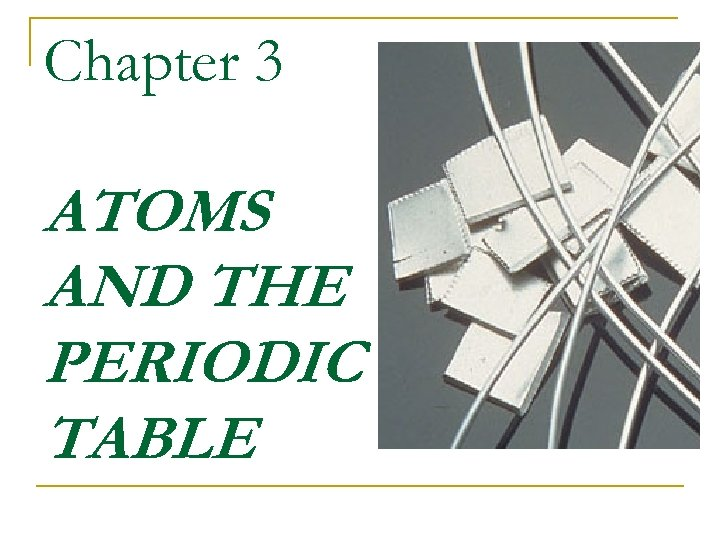Chapter 3 ATOMS AND THE PERIODIC TABLE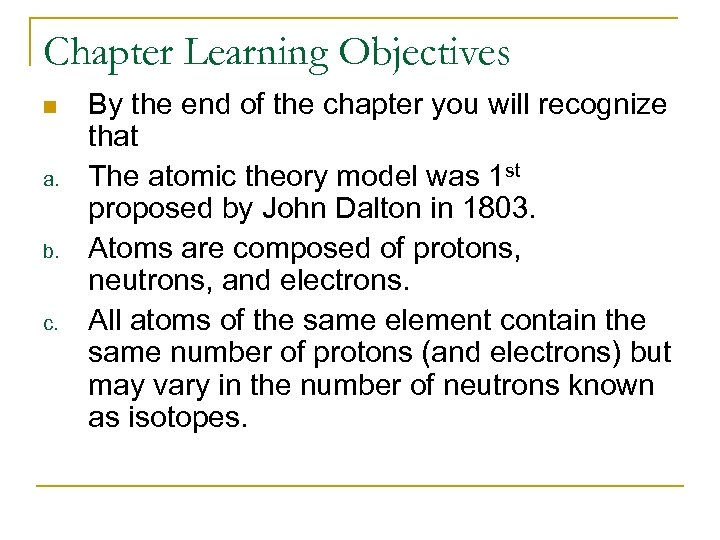Chapter Learning Objectives n a. b. c. By the end of the chapter you will recognize that The atomic theory model was 1 st proposed by John Dalton in 1803. Atoms are composed of protons, neutrons, and electrons. All atoms of the same element contain the same number of protons (and electrons) but may vary in the number of neutrons known as isotopes.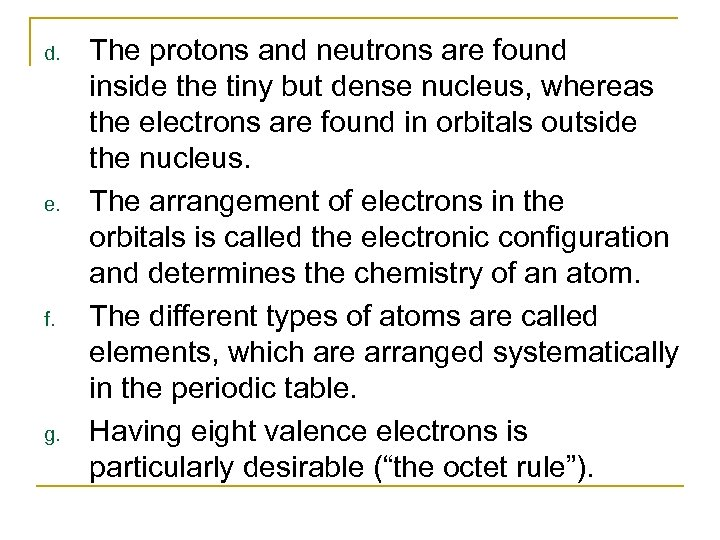d. e. f. g. The protons and neutrons are found inside the tiny but dense nucleus, whereas the electrons are found in orbitals outside the nucleus. The arrangement of electrons in the orbitals is called the electronic configuration and determines the chemistry of an atom. The different types of atoms are called elements, which are arranged systematically in the periodic table. Having eight valence electrons is particularly desirable (“the octet rule”).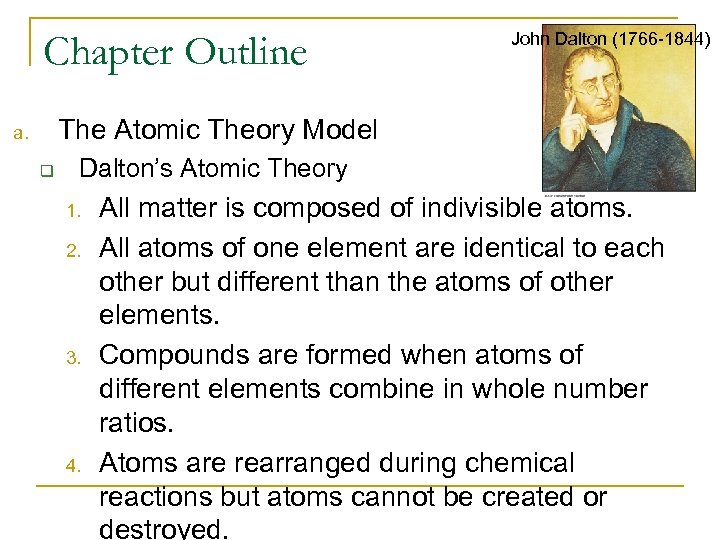Chapter Outline John Dalton (1766 -1844) The Atomic Theory Model a. q Dalton’s Atomic Theory 1. 2. 3. 4. All matter is composed of indivisible atoms. All atoms of one element are identical to each other but different than the atoms of other elements. Compounds are formed when atoms of different elements combine in whole number ratios. Atoms are rearranged during chemical reactions but atoms cannot be created or destroyed.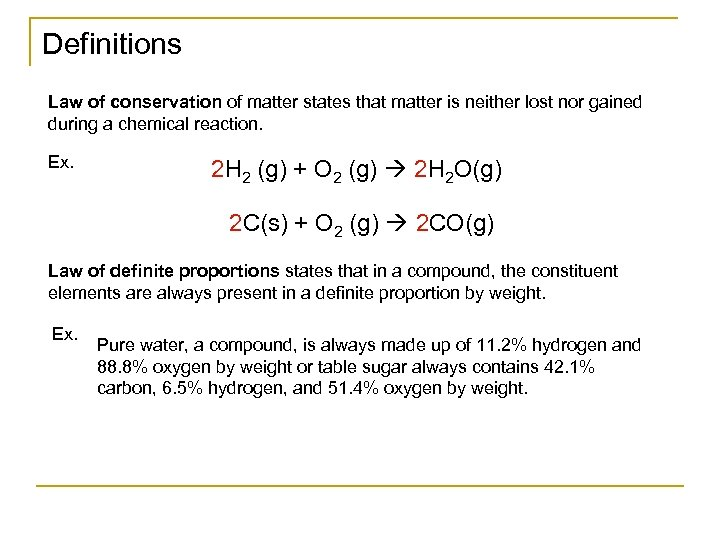Definitions Law of conservation of matter states that matter is neither lost nor gained during a chemical reaction. Ex. 2 H 2 (g) + O 2 (g) 2 H 2 O(g) 2 C(s) + O 2 (g) 2 CO(g) Law of definite proportions states that in a compound, the constituent elements are always present in a definite proportion by weight. Ex. Pure water, a compound, is always made up of 11. 2% hydrogen and 88. 8% oxygen by weight or table sugar always contains 42. 1% carbon, 6. 5% hydrogen, and 51. 4% oxygen by weight.Run the following web animations/movies. 3. 1: Conservation of Matter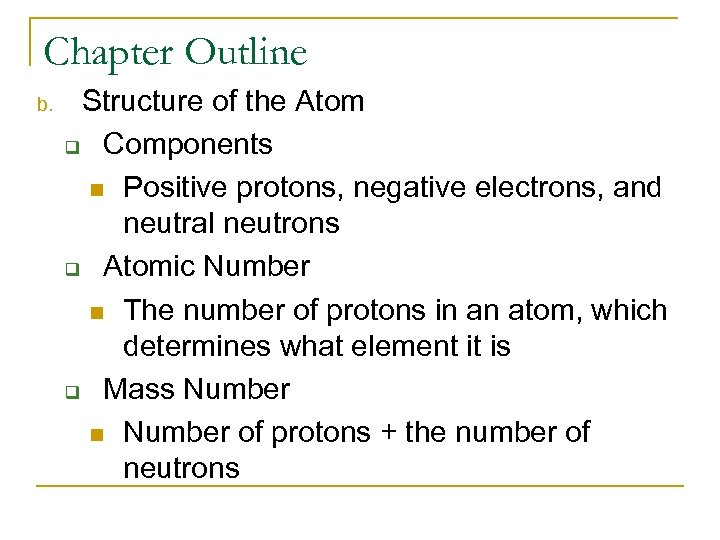Chapter Outline b. Structure of the Atom q Components n Positive protons, negative electrons, and neutral neutrons q Atomic Number n The number of protons in an atom, which determines what element it is q Mass Number n Number of protons + the number of neutrons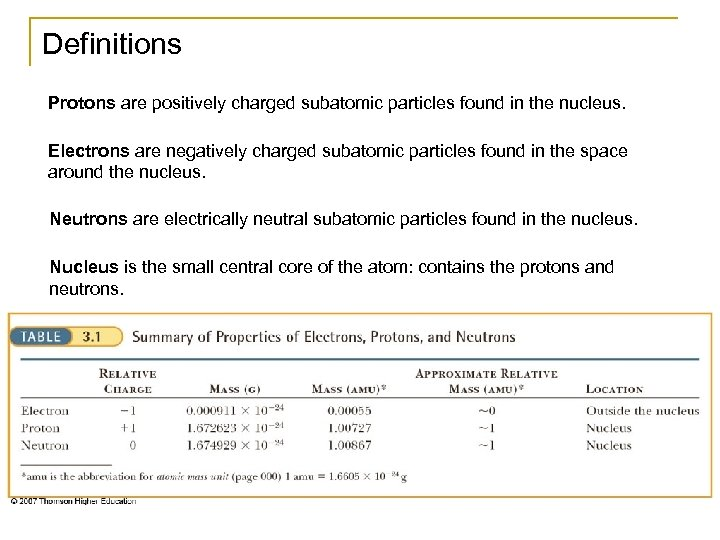Definitions Protons are positively charged subatomic particles found in the nucleus. Electrons are negatively charged subatomic particles found in the space around the nucleus. Neutrons are electrically neutral subatomic particles found in the nucleus. Nucleus is the small central core of the atom: contains the protons and neutrons.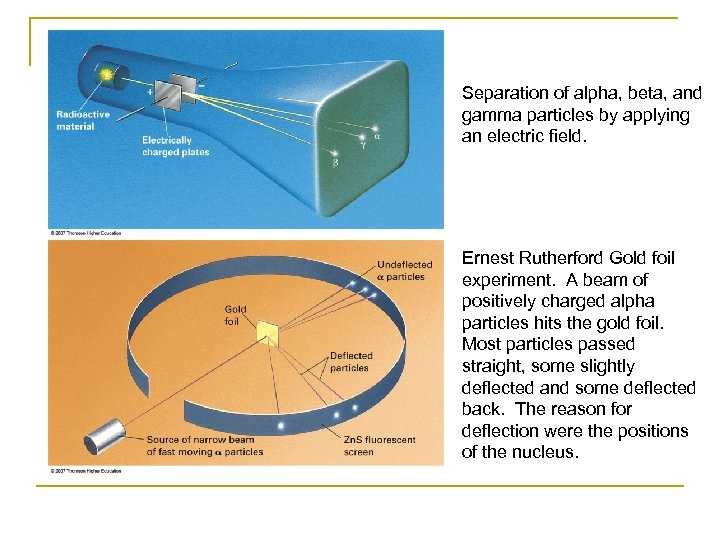Separation of alpha, beta, and gamma particles by applying an electric field. Ernest Rutherford Gold foil experiment. A beam of positively charged alpha particles hits the gold foil. Most particles passed straight, some slightly deflected and some deflected back. The reason for deflection were the positions of the nucleus.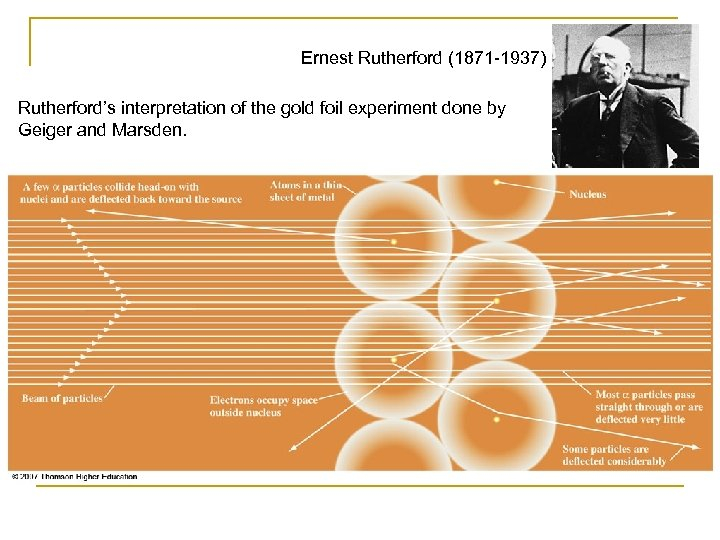Ernest Rutherford (1871 -1937) Rutherford’s interpretation of the gold foil experiment done by Geiger and Marsden.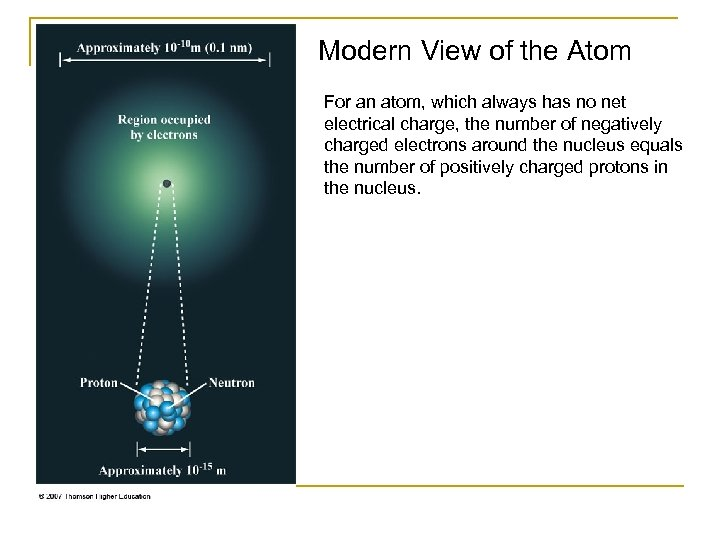Modern View of the Atom For an atom, which always has no net electrical charge, the number of negatively charged electrons around the nucleus equals the number of positively charged protons in the nucleus.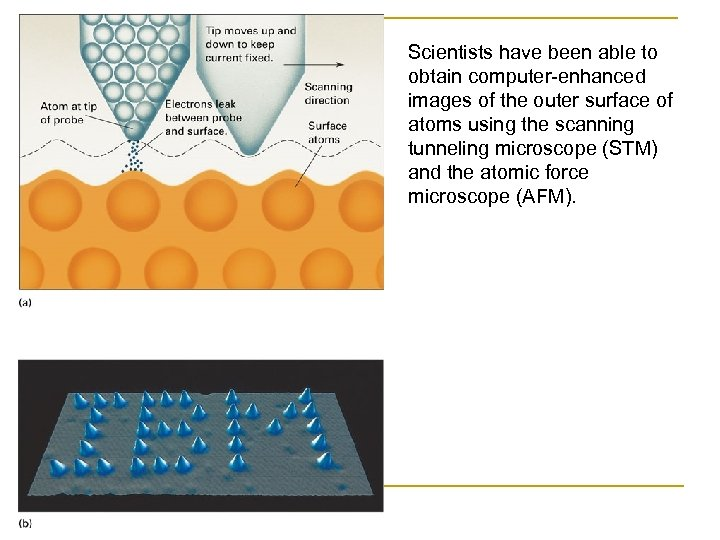Scientists have been able to obtain computer-enhanced images of the outer surface of atoms using the scanning tunneling microscope (STM) and the atomic force microscope (AFM).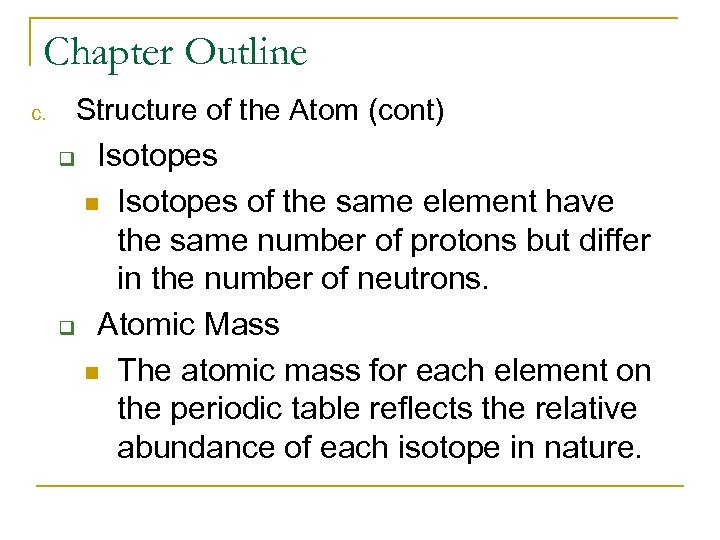Chapter Outline Structure of the Atom (cont) c. Isotopes n Isotopes of the same element have the same number of protons but differ in the number of neutrons. q Atomic Mass n The atomic mass for each element on the periodic table reflects the relative abundance of each isotope in nature. q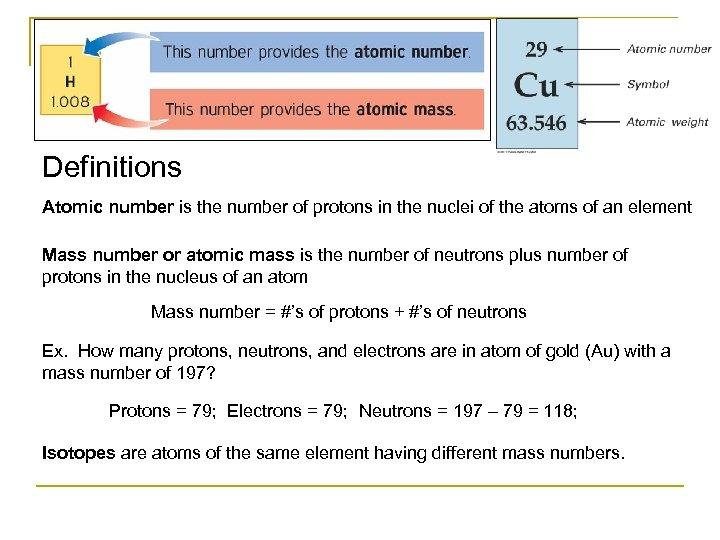Definitions Atomic number is the number of protons in the nuclei of the atoms of an element Mass number or atomic mass is the number of neutrons plus number of protons in the nucleus of an atom Mass number = #’s of protons + #’s of neutrons Ex. How many protons, neutrons, and electrons are in atom of gold (Au) with a mass number of 197? Protons = 79; Electrons = 79; Neutrons = 197 – 79 = 118; Isotopes are atoms of the same element having different mass numbers.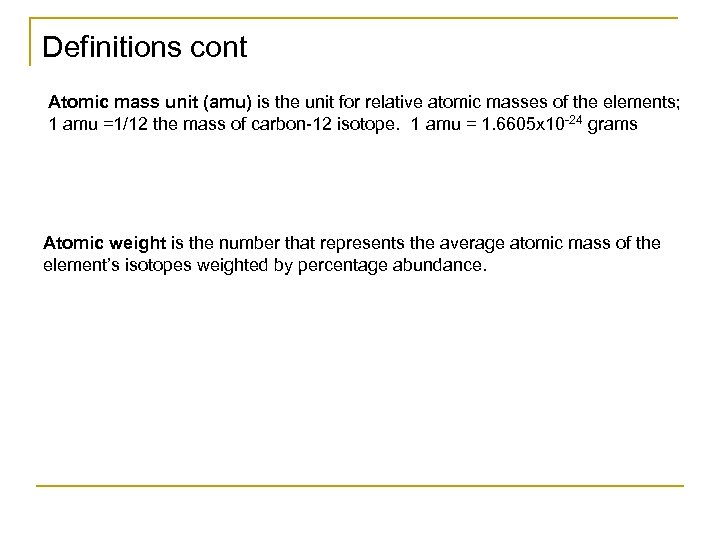Definitions cont Atomic mass unit (amu) is the unit for relative atomic masses of the elements; 1 amu =1/12 the mass of carbon-12 isotope. 1 amu = 1. 6605 x 10 -24 grams Atomic weight is the number that represents the average atomic mass of the element’s isotopes weighted by percentage abundance.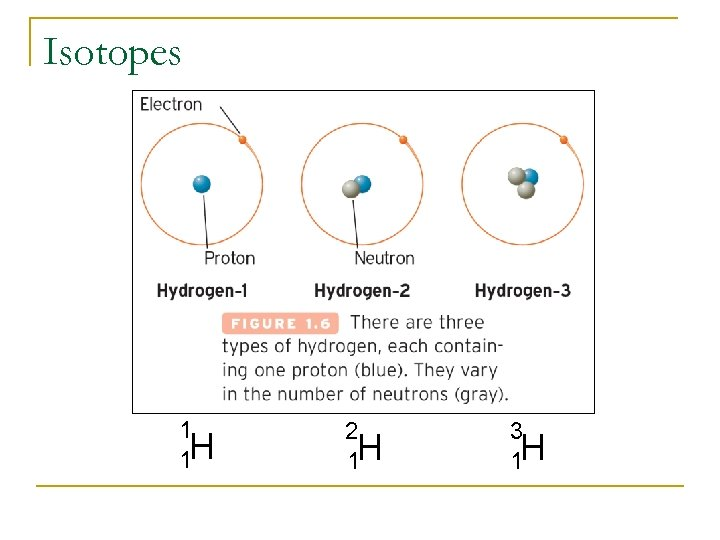Isotopes 1 1 H 2 1 H 3 1 H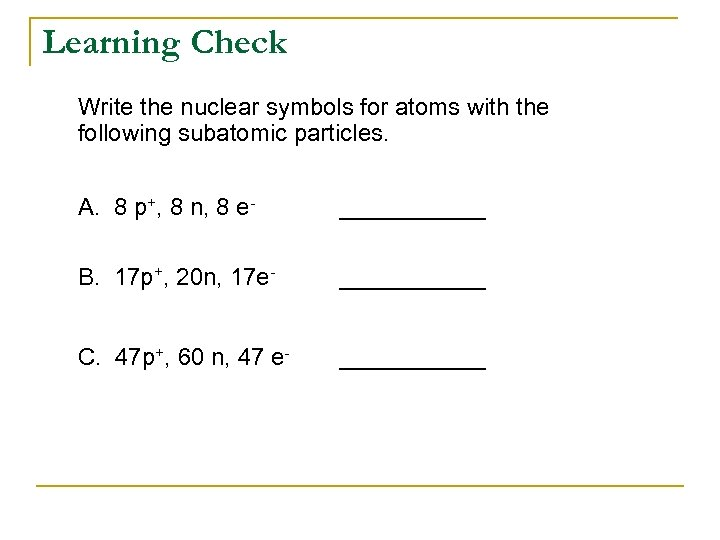Learning Check Write the nuclear symbols for atoms with the following subatomic particles. A. 8 p+, 8 n, 8 e- ______ B. 17 p+, 20 n, 17 e- ______ C. 47 p+, 60 n, 47 e- ______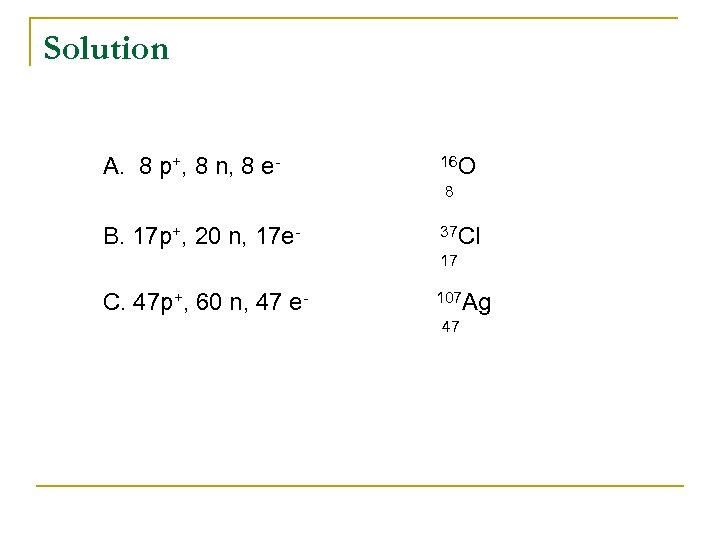Solution A. 8 p+, 8 n, 8 e- 16 O 8 B. 17 p+, 20 n, 17 e- 37 Cl 17 C. 47 p+, 60 n, 47 e- 107 Ag 47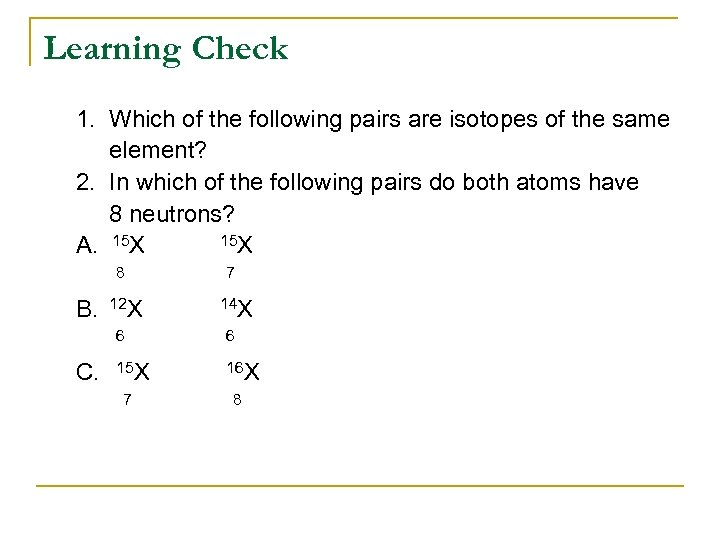Learning Check 1. Which of the following pairs are isotopes of the same element? 2. In which of the following pairs do both atoms have 8 neutrons? 15 X A. 15 X 8 B. 12 X 7 14 X 6 C. 6 15 X 16 X 7 8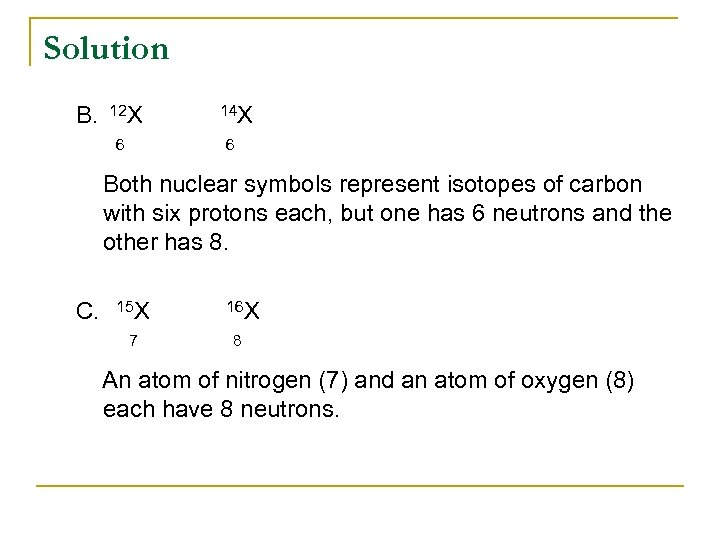Solution B. 12 X 6 14 X 6 Both nuclear symbols represent isotopes of carbon with six protons each, but one has 6 neutrons and the other has 8. C. 15 X 7 16 X 8 An atom of nitrogen (7) and an atom of oxygen (8) each have 8 neutrons.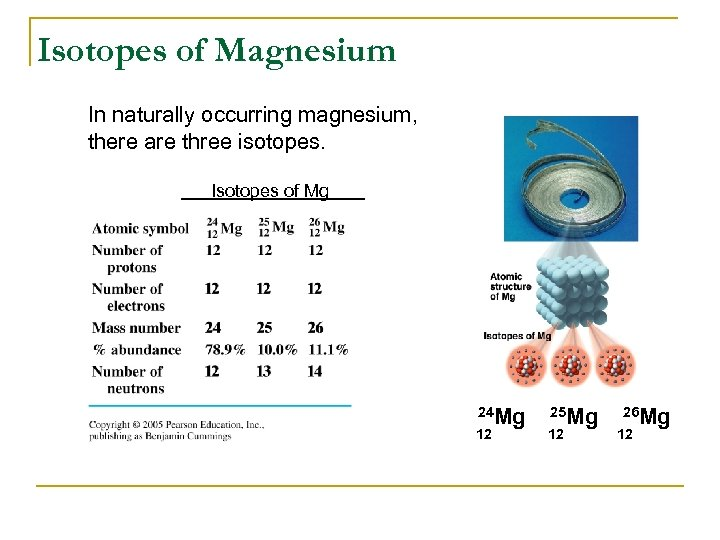Isotopes of Magnesium In naturally occurring magnesium, there are three isotopes. Isotopes of Mg 24 Mg 12 25 Mg 12 26 Mg 12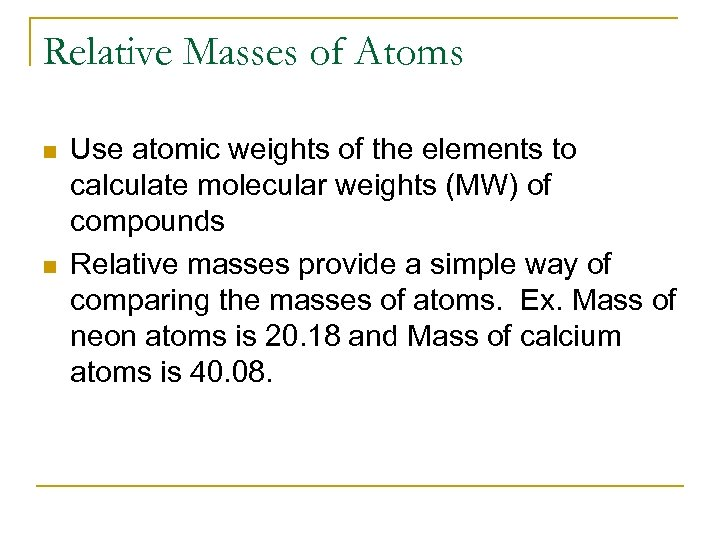Relative Masses of Atoms n n Use atomic weights of the elements to calculate molecular weights (MW) of compounds Relative masses provide a simple way of comparing the masses of atoms. Ex. Mass of neon atoms is 20. 18 and Mass of calcium atoms is 40. 08.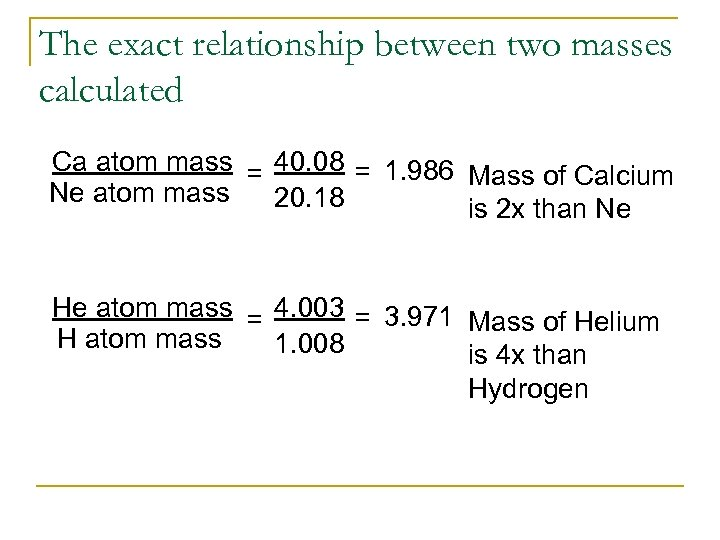The exact relationship between two masses calculated Ca atom mass = 40. 08 = 1. 986 Mass of Calcium Ne atom mass 20. 18 is 2 x than Ne He atom mass = 4. 003 = 3. 971 Mass of Helium H atom mass 1. 008 is 4 x than Hydrogen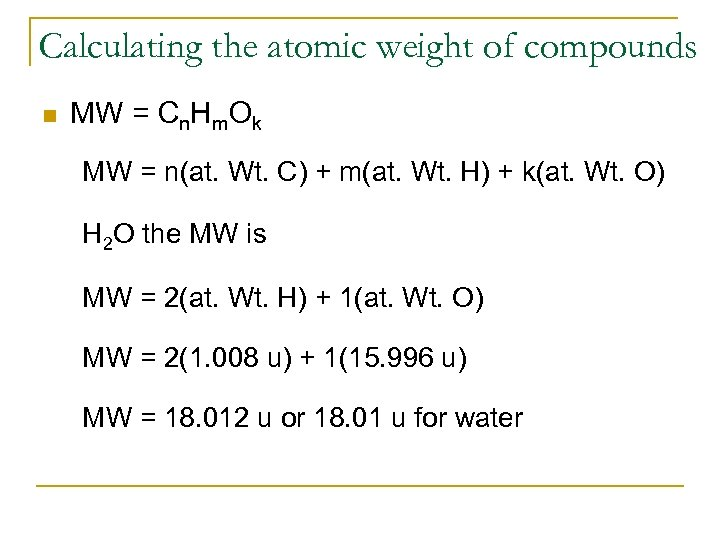Calculating the atomic weight of compounds n MW = Cn. Hm. Ok MW = n(at. Wt. C) + m(at. Wt. H) + k(at. Wt. O) H 2 O the MW is MW = 2(at. Wt. H) + 1(at. Wt. O) MW = 2(1. 008 u) + 1(15. 996 u) MW = 18. 012 u or 18. 01 u for water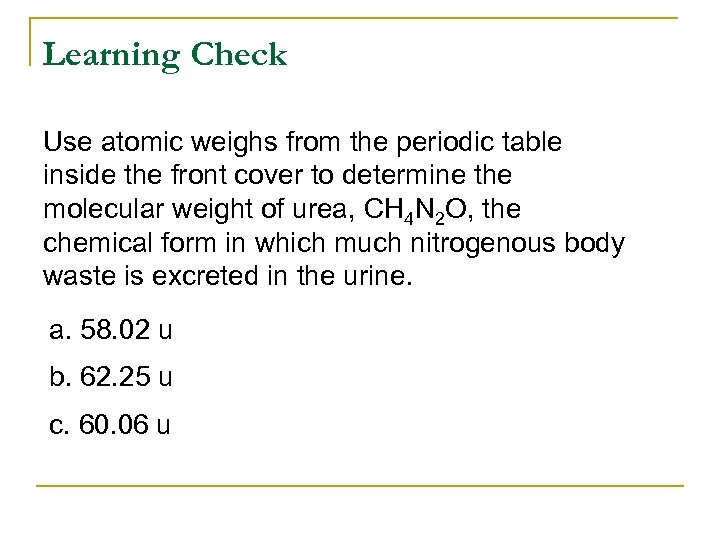Learning Check Use atomic weighs from the periodic table inside the front cover to determine the molecular weight of urea, CH 4 N 2 O, the chemical form in which much nitrogenous body waste is excreted in the urine. a. 58. 02 u b. 62. 25 u c. 60. 06 u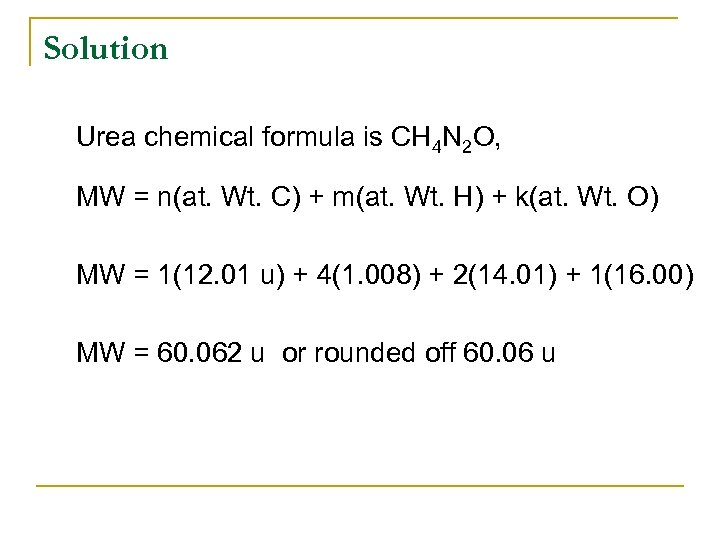Solution Urea chemical formula is CH 4 N 2 O, MW = n(at. Wt. C) + m(at. Wt. H) + k(at. Wt. O) MW = 1(12. 01 u) + 4(1. 008) + 2(14. 01) + 1(16. 00) MW = 60. 062 u or rounded off 60. 06 u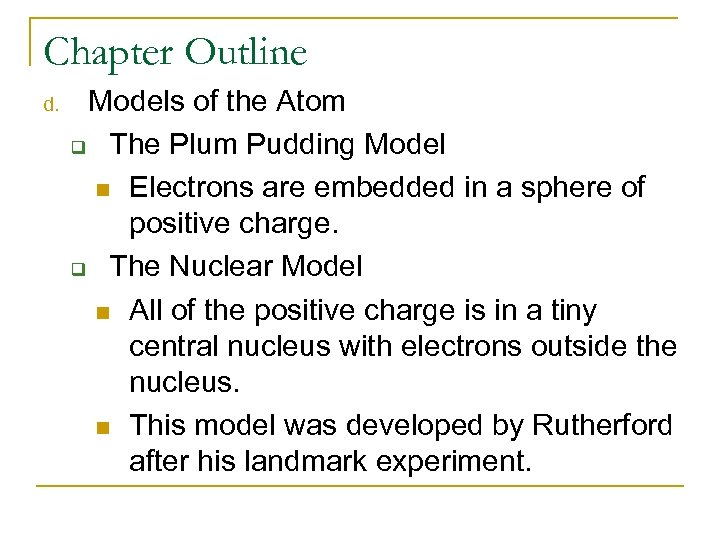Chapter Outline d. Models of the Atom q The Plum Pudding Model n Electrons are embedded in a sphere of positive charge. q The Nuclear Model n All of the positive charge is in a tiny central nucleus with electrons outside the nucleus. n This model was developed by Rutherford after his landmark experiment.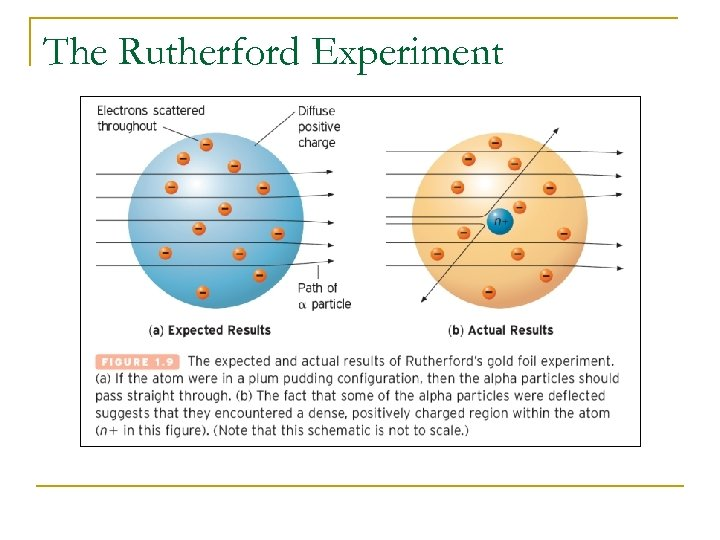The Rutherford Experiment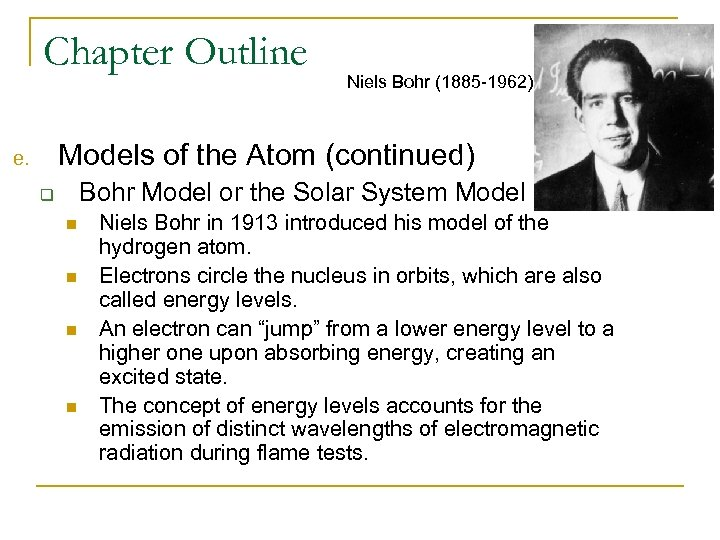Chapter Outline Niels Bohr (1885 -1962) Models of the Atom (continued) e. Bohr Model or the Solar System Model q n n Niels Bohr in 1913 introduced his model of the hydrogen atom. Electrons circle the nucleus in orbits, which are also called energy levels. An electron can “jump” from a lower energy level to a higher one upon absorbing energy, creating an excited state. The concept of energy levels accounts for the emission of distinct wavelengths of electromagnetic radiation during flame tests.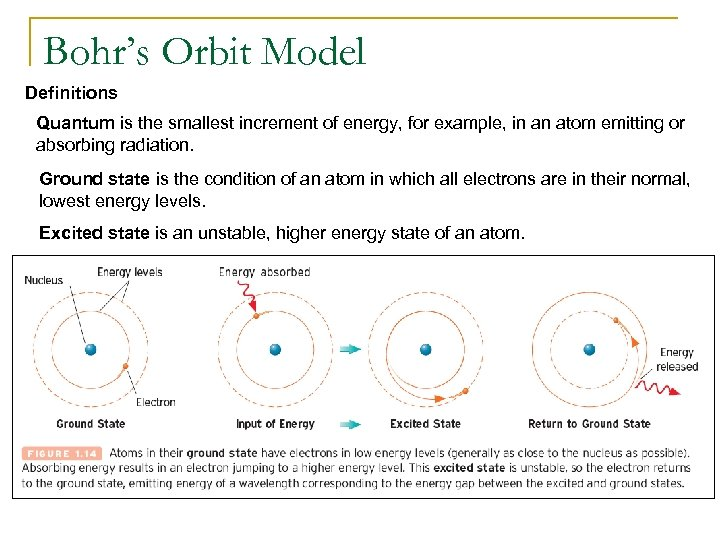Bohr’s Orbit Model Definitions Quantum is the smallest increment of energy, for example, in an atom emitting or absorbing radiation. Ground state is the condition of an atom in which all electrons are in their normal, lowest energy levels. Excited state is an unstable, higher energy state of an atom.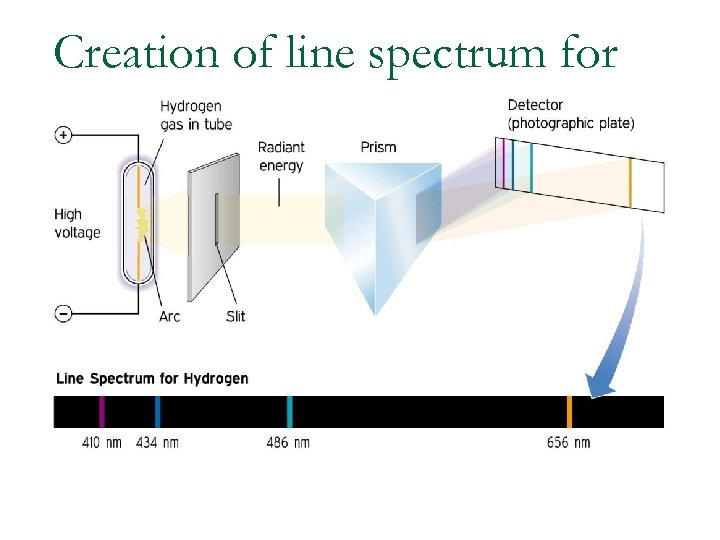Creation of line spectrum for hydrogen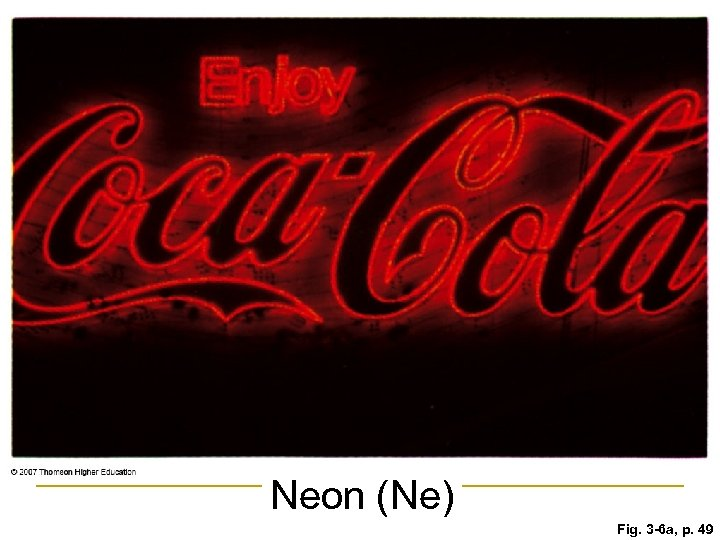Neon (Ne) Fig. 3 -6 a, p. 49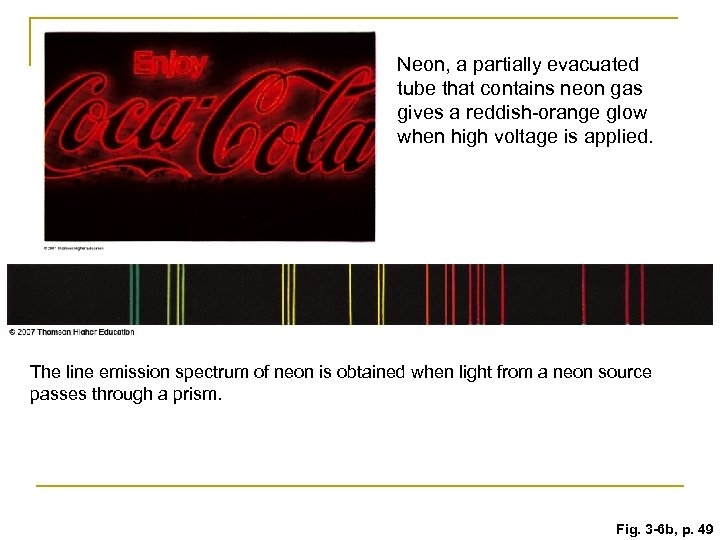Neon, a partially evacuated tube that contains neon gas gives a reddish-orange glow when high voltage is applied. The line emission spectrum of neon is obtained when light from a neon source passes through a prism. Fig. 3 -6 b, p. 49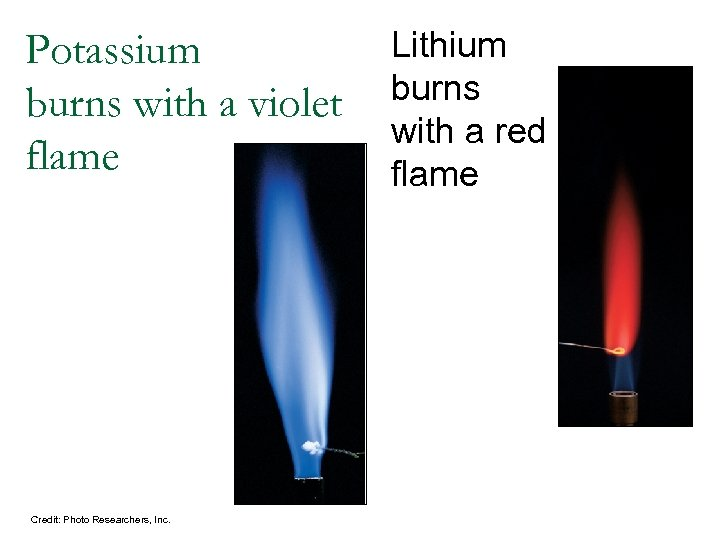Potassium burns with a violet flame Credit: Photo Researchers, Inc. Lithium burns with a red flameRun the following web animations/movies. 3. 2: Atomic Line Spectra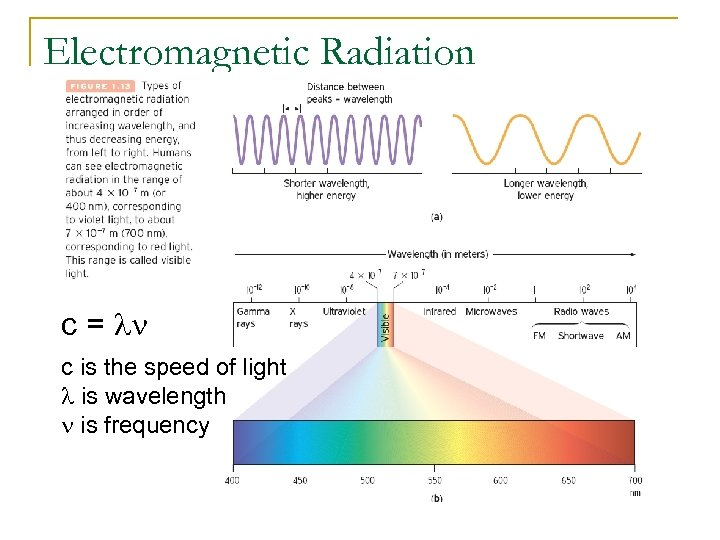Electromagnetic Radiation c = ln c is the speed of light l is wavelength n is frequency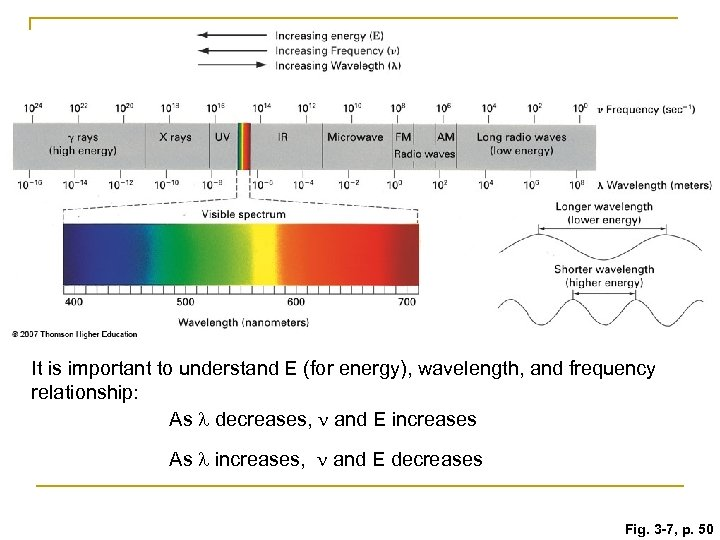It is important to understand E (for energy), wavelength, and frequency relationship: As l decreases, n and E increases As l increases, n and E decreases Fig. 3 -7, p. 50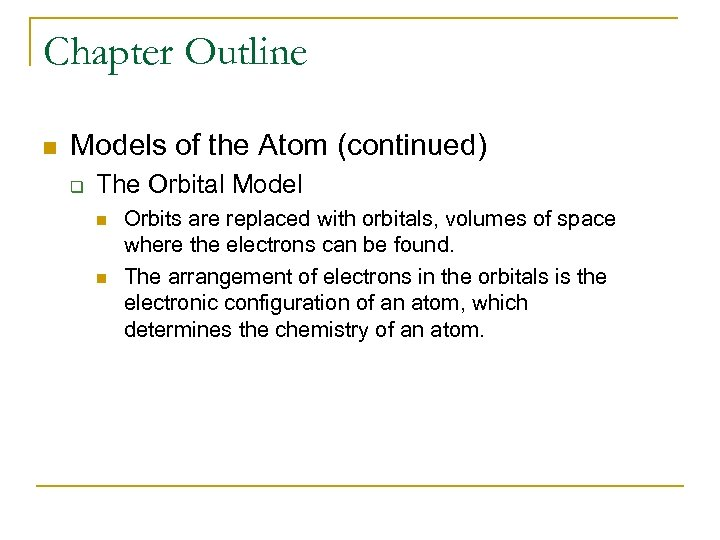Chapter Outline n Models of the Atom (continued) q The Orbital Model n n Orbits are replaced with orbitals, volumes of space where the electrons can be found. The arrangement of electrons in the orbitals is the electronic configuration of an atom, which determines the chemistry of an atom.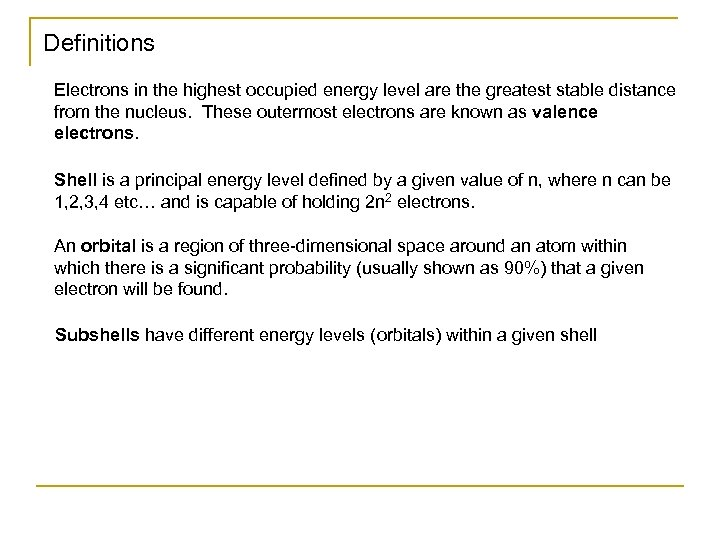Definitions Electrons in the highest occupied energy level are the greatest stable distance from the nucleus. These outermost electrons are known as valence electrons. Shell is a principal energy level defined by a given value of n, where n can be 1, 2, 3, 4 etc… and is capable of holding 2 n 2 electrons. An orbital is a region of three-dimensional space around an atom within which there is a significant probability (usually shown as 90%) that a given electron will be found. Subshells have different energy levels (orbitals) within a given shell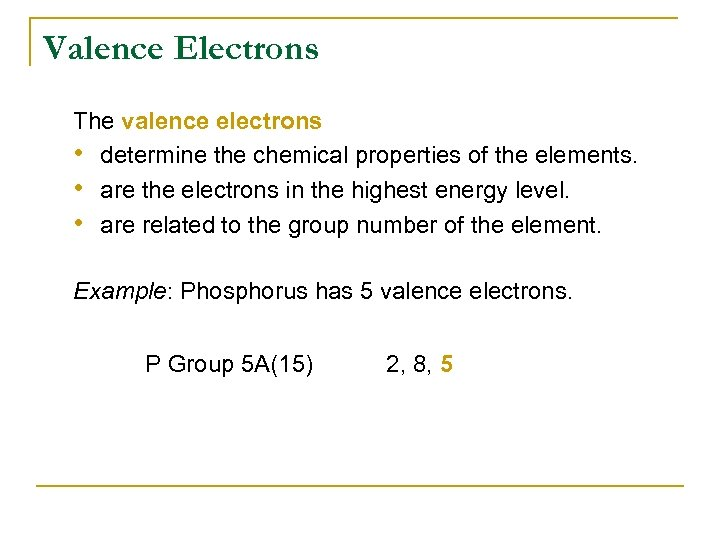Valence Electrons The valence electrons • determine the chemical properties of the elements. • are the electrons in the highest energy level. • are related to the group number of the element. Example: Phosphorus has 5 valence electrons. P Group 5 A(15) 2, 8, 5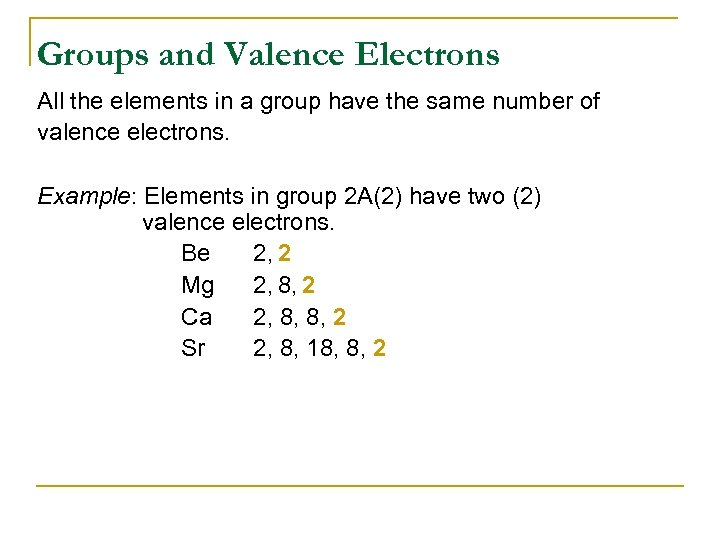Groups and Valence Electrons All the elements in a group have the same number of valence electrons. Example: Elements in group 2 A(2) have two (2) valence electrons. Be 2, 2 Mg 2, 8, 2 Ca 2, 8, 8, 2 Sr 2, 8, 18, 8, 2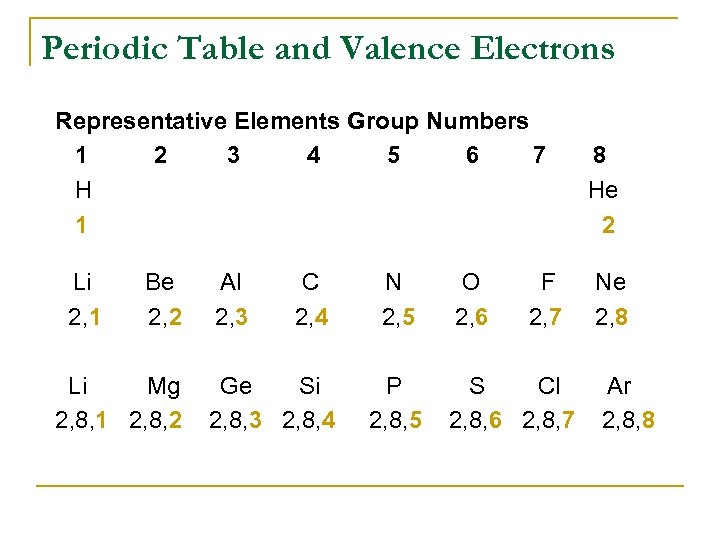Periodic Table and Valence Electrons Representative Elements Group Numbers 1 2 3 4 5 6 7 H 1 Li 2, 1 Be 2, 2 Li Mg 2, 8, 1 2, 8, 2 Al 2, 3 C 2, 4 N 2, 5 Ge Si 2, 8, 3 2, 8, 4 P 2, 8, 5 O 2, 6 F 2, 7 S Cl 2, 8, 6 2, 8, 7 8 He 2 Ne 2, 8 Ar 2, 8, 8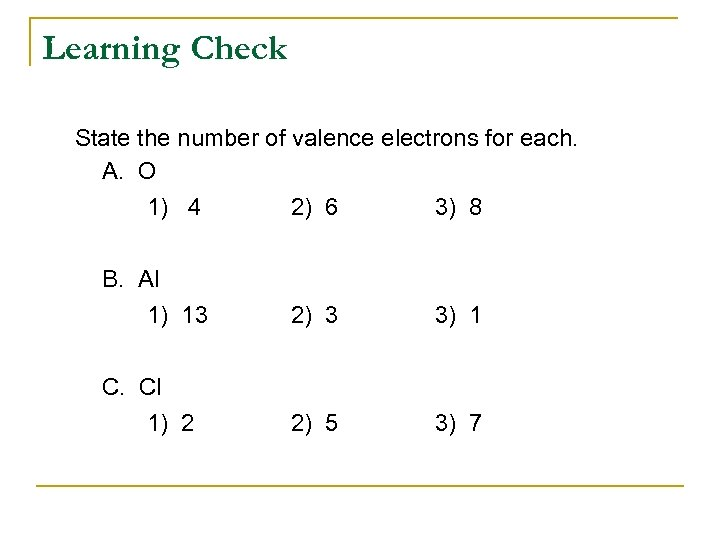Learning Check State the number of valence electrons for each. A. O 1) 4 2) 6 3) 8 B. Al 1) 13 2) 3 3) 1 C. Cl 1) 2 2) 5 3) 7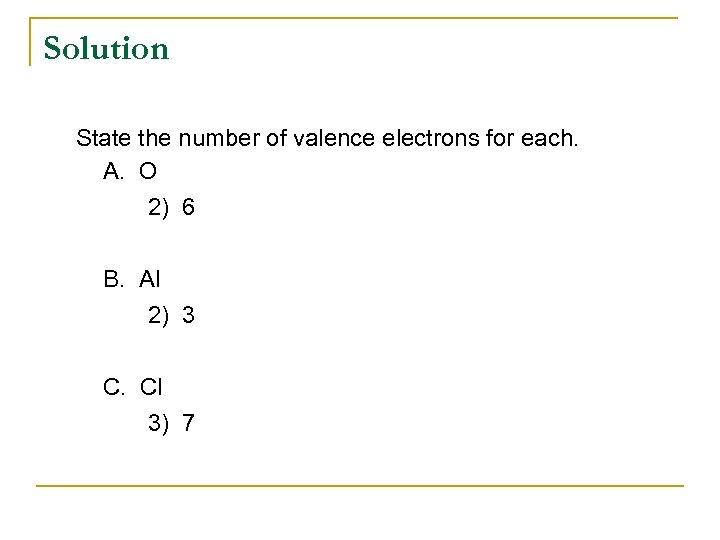Solution State the number of valence electrons for each. A. O 2) 6 B. Al 2) 3 C. Cl 3) 7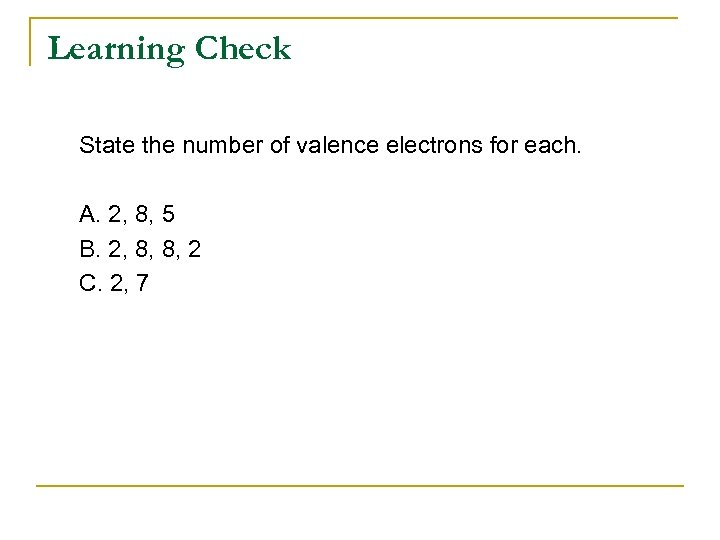Learning Check State the number of valence electrons for each. A. 2, 8, 5 B. 2, 8, 8, 2 C. 2, 7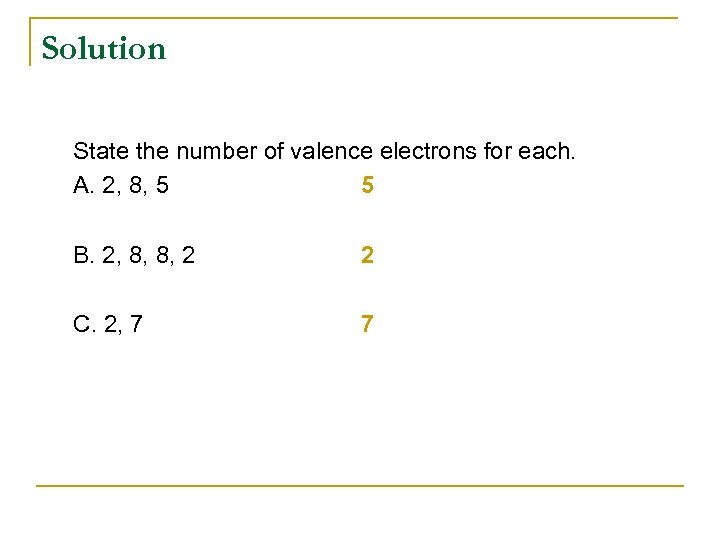Solution State the number of valence electrons for each. A. 2, 8, 5 5 B. 2, 8, 8, 2 2 C. 2, 7 7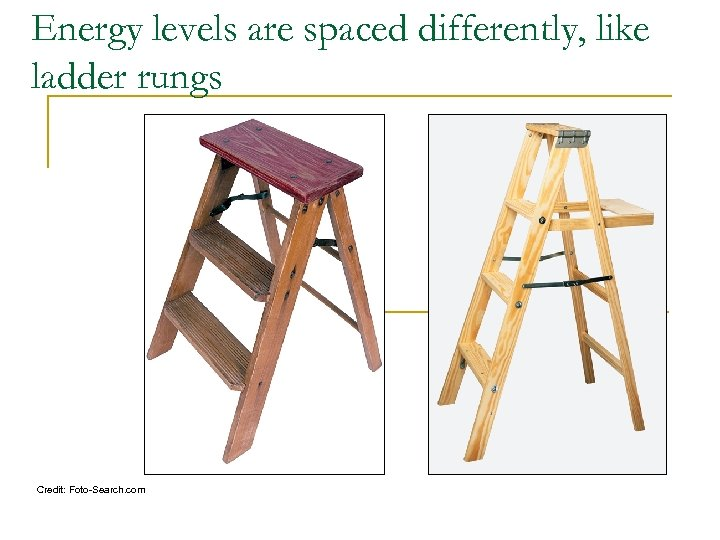Energy levels are spaced differently, like ladder rungs Credit: Foto-Search. com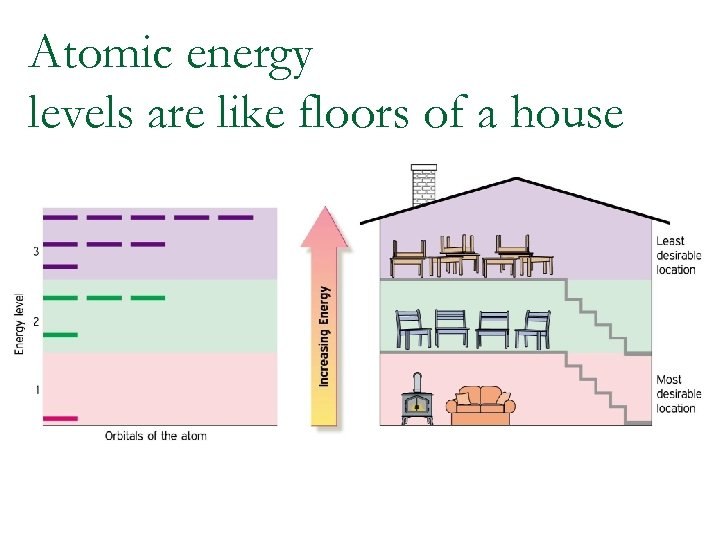Atomic energy levels are like floors of a house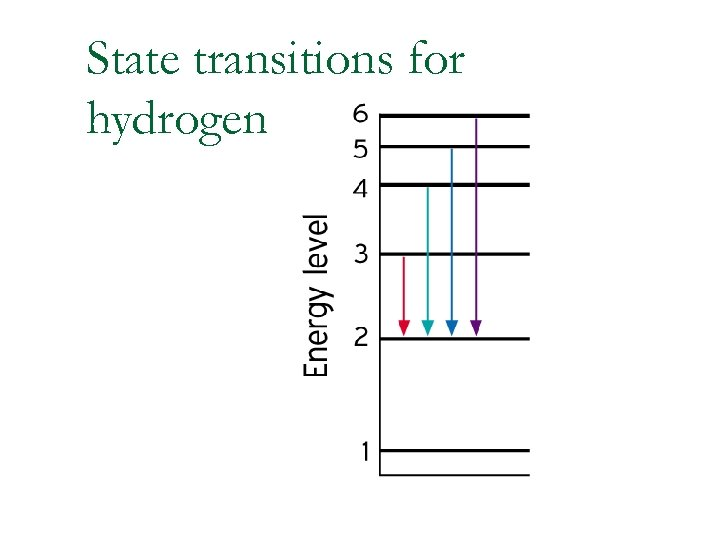State transitions for hydrogen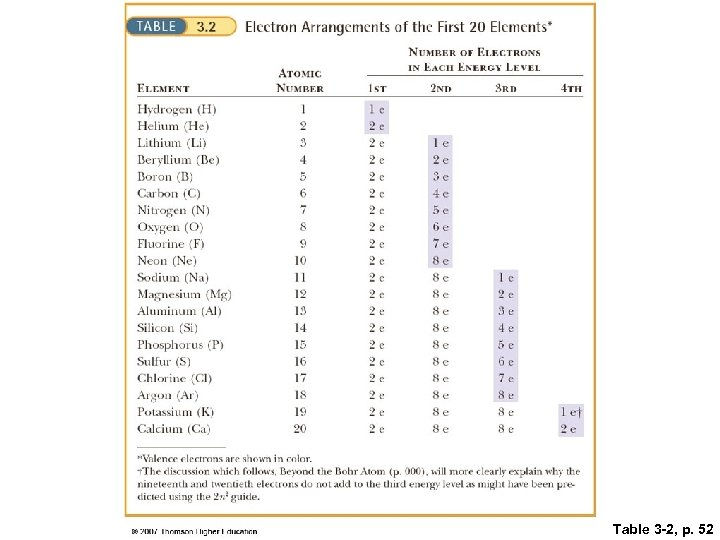Table 3 -2, p. 52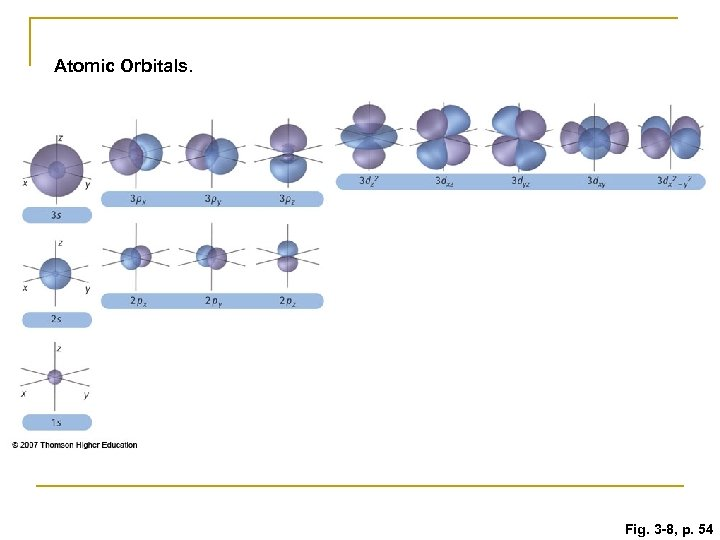Atomic Orbitals. Fig. 3 -8, p. 54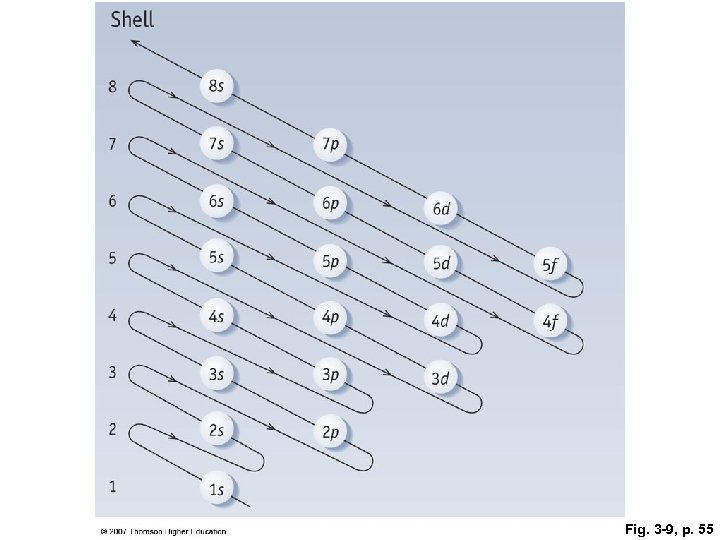Fig. 3 -9, p. 55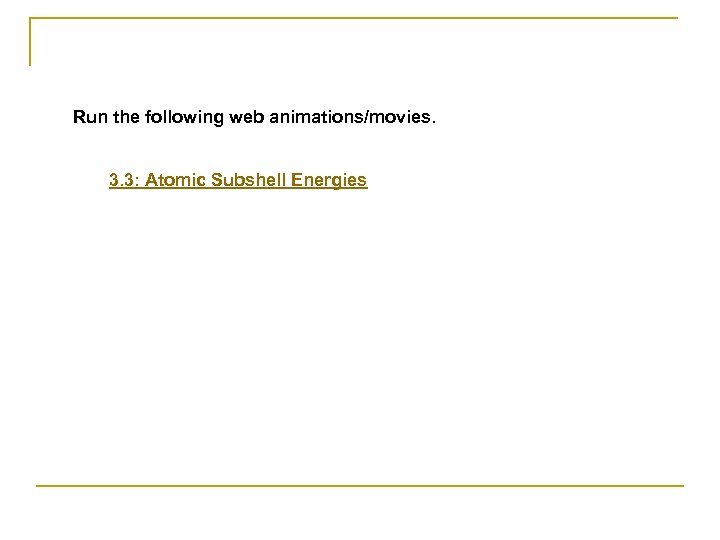Run the following web animations/movies. 3. 3: Atomic Subshell Energies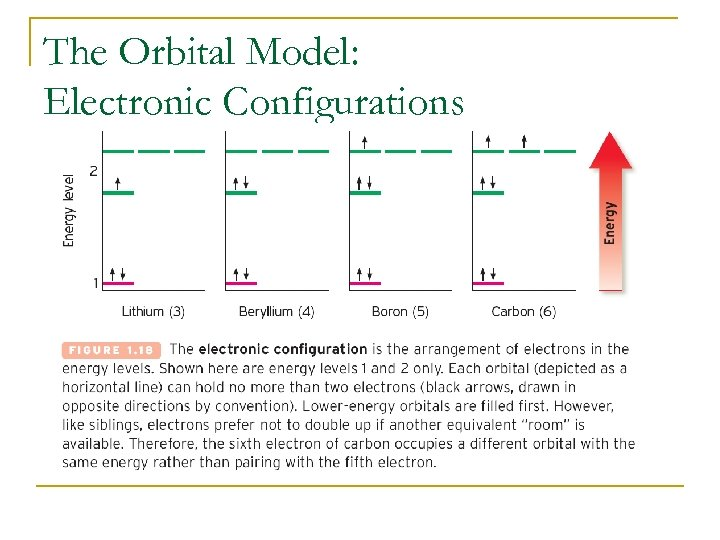The Orbital Model: Electronic Configurations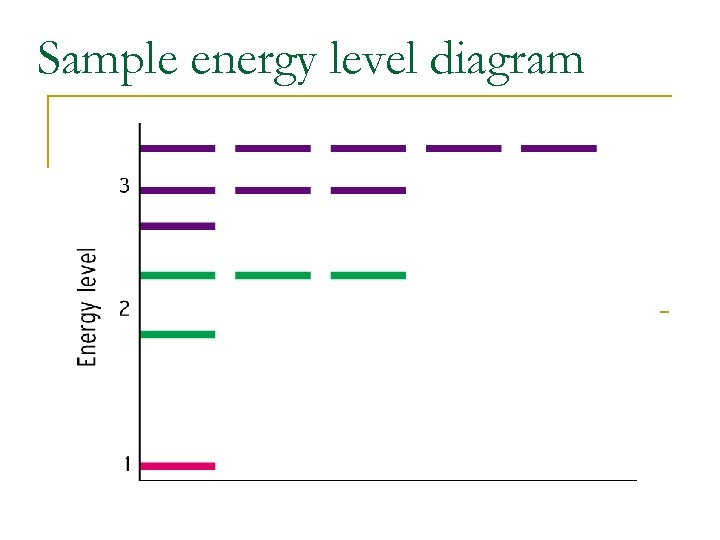Sample energy level diagram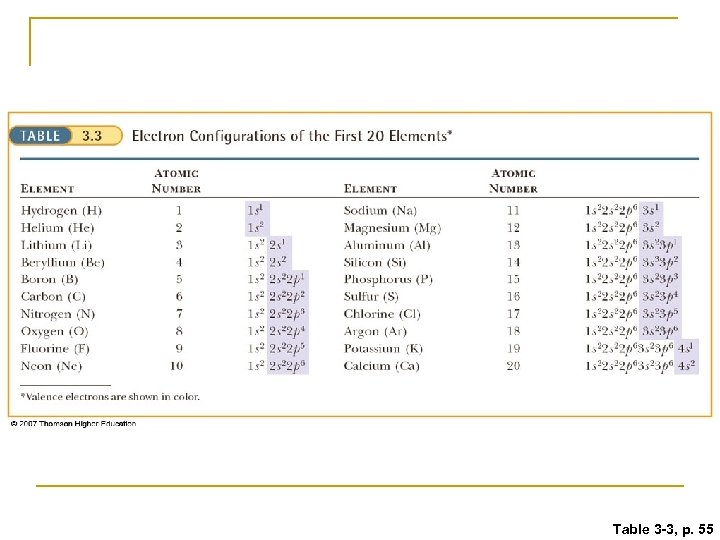Table 3 -3, p. 55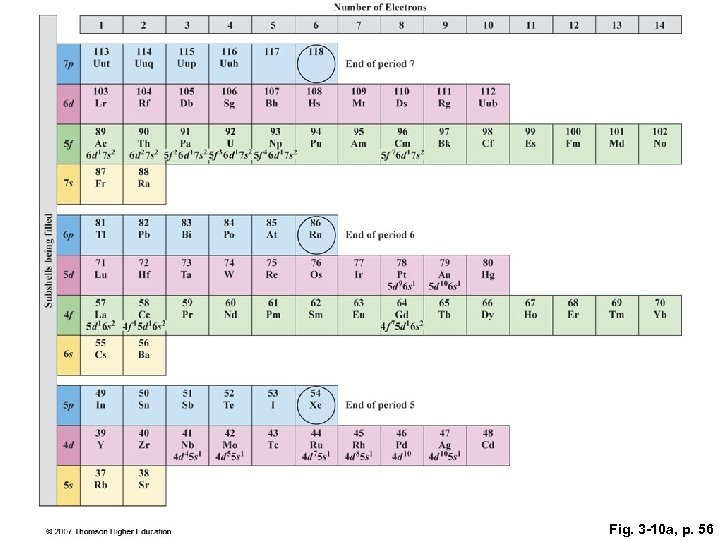Fig. 3 -10 a, p. 56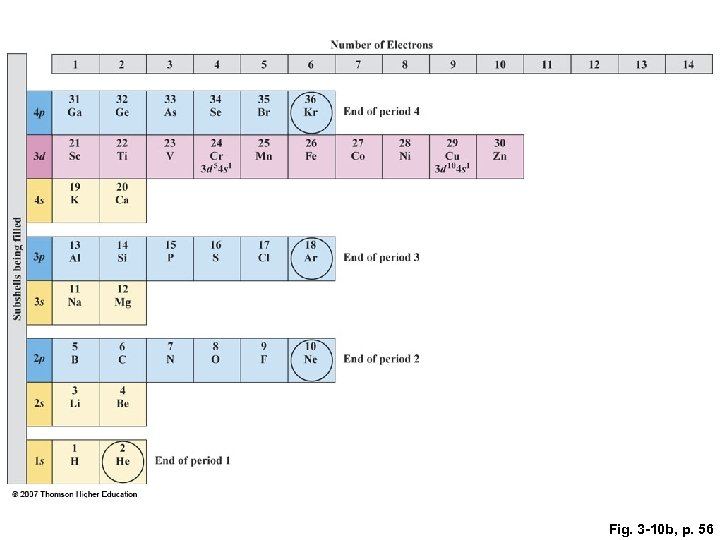Fig. 3 -10 b, p. 56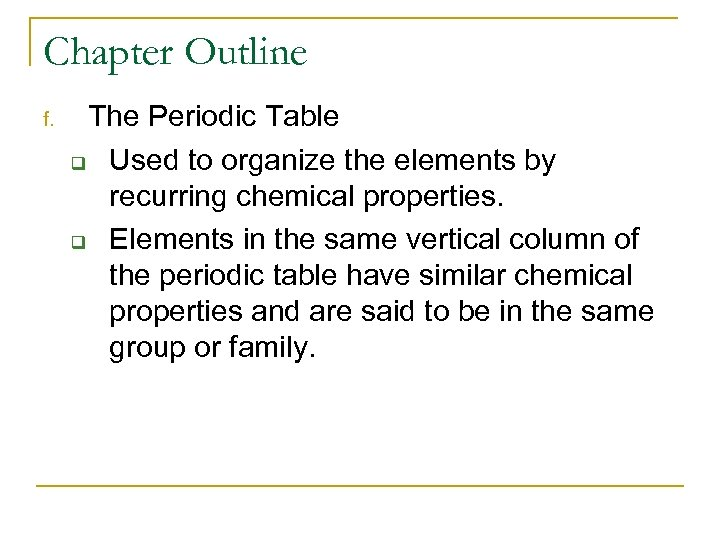Chapter Outline f. The Periodic Table q Used to organize the elements by recurring chemical properties. q Elements in the same vertical column of the periodic table have similar chemical properties and are said to be in the same group or family.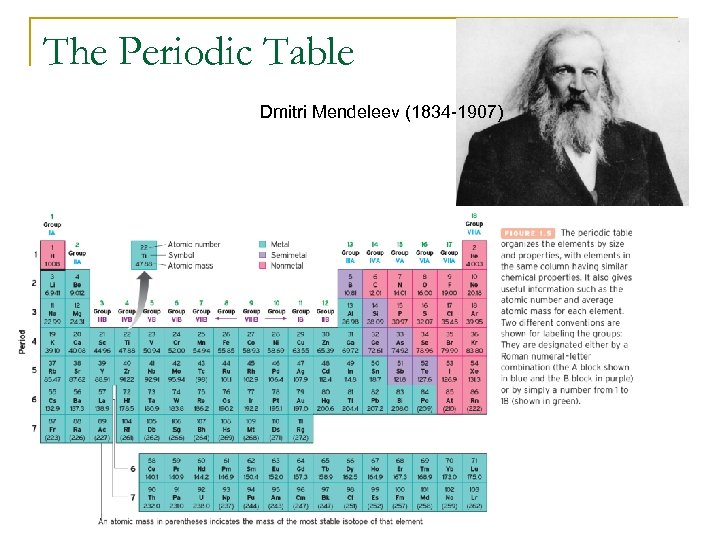The Periodic Table Dmitri Mendeleev (1834 -1907)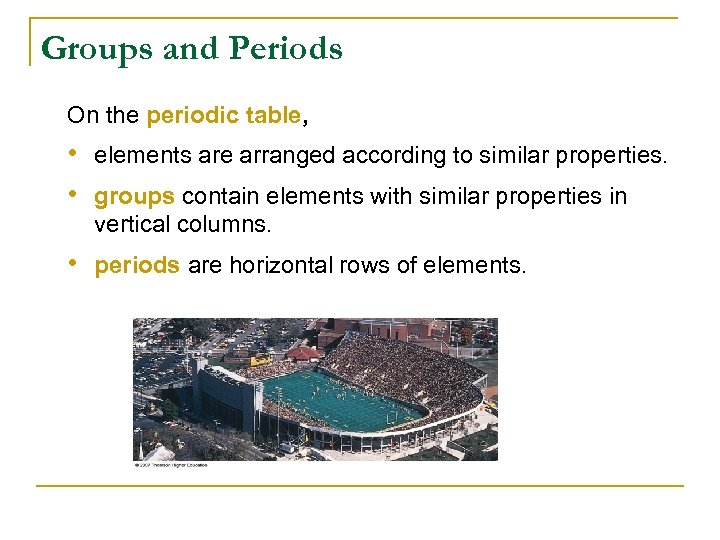Groups and Periods On the periodic table, • elements are arranged according to similar properties. • groups contain elements with similar properties in vertical columns. • periods are horizontal rows of elements.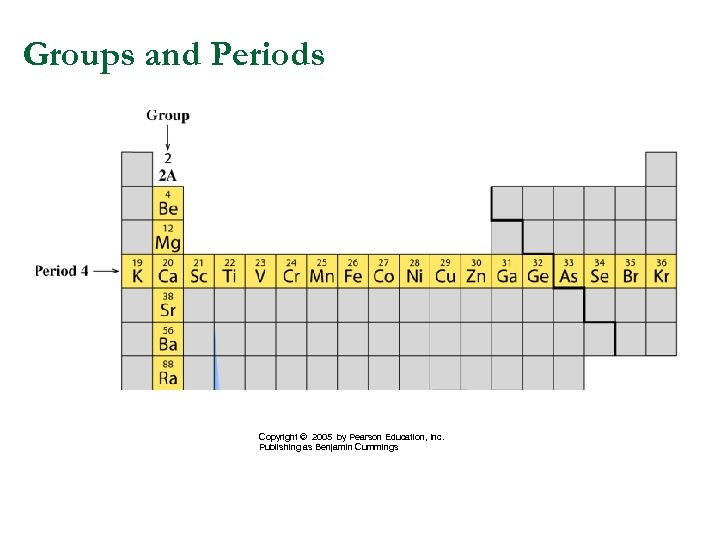Groups and Periods Copyright © 2005 by Pearson Education, Inc. Publishing as Benjamin Cummings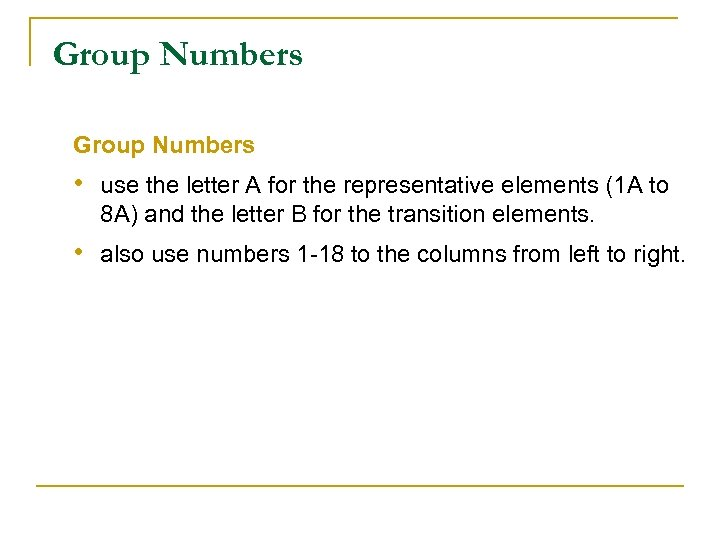Group Numbers • use the letter A for the representative elements (1 A to 8 A) and the letter B for the transition elements. • also use numbers 1 -18 to the columns from left to right.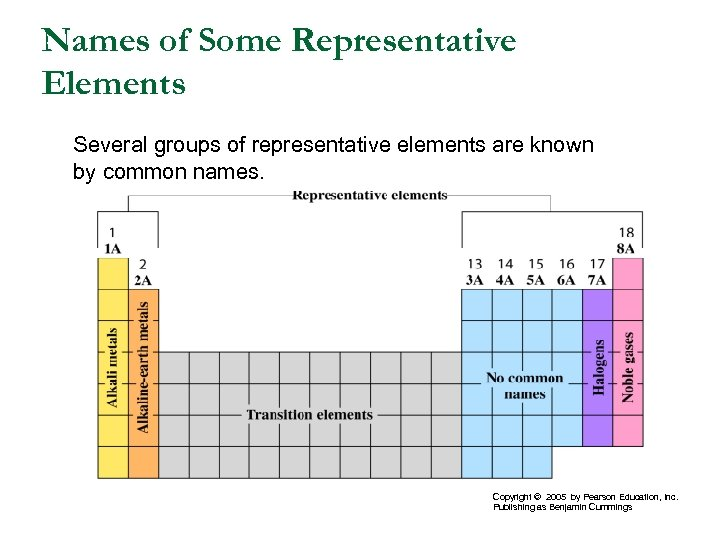Names of Some Representative Elements Several groups of representative elements are known by common names. Copyright © 2005 by Pearson Education, Inc. Publishing as Benjamin Cummings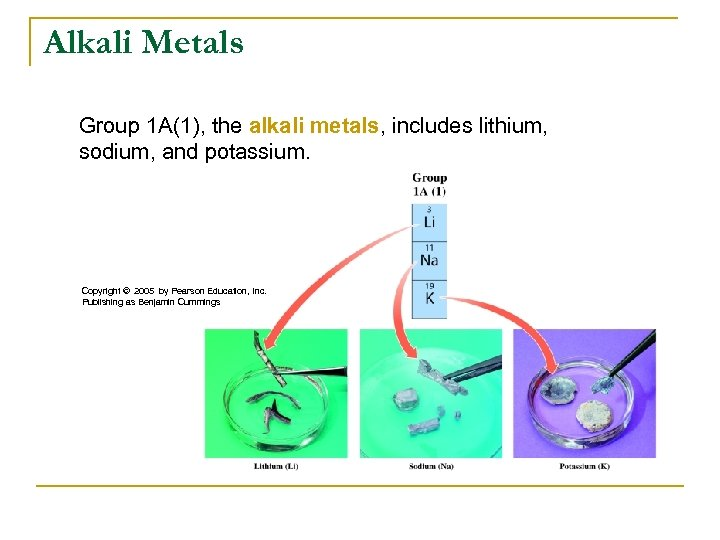Alkali Metals Group 1 A(1), the alkali metals, includes lithium, sodium, and potassium. Copyright © 2005 by Pearson Education, Inc. Publishing as Benjamin Cummings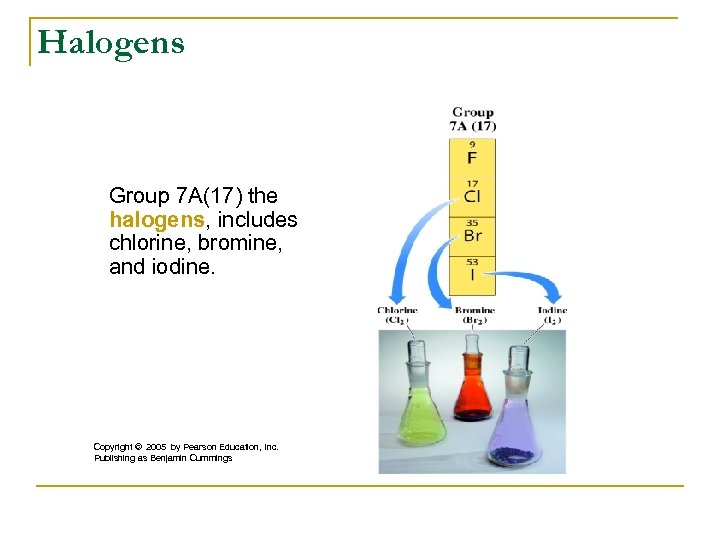Halogens Group 7 A(17) the halogens, includes chlorine, bromine, and iodine. Copyright © 2005 by Pearson Education, Inc. Publishing as Benjamin Cummings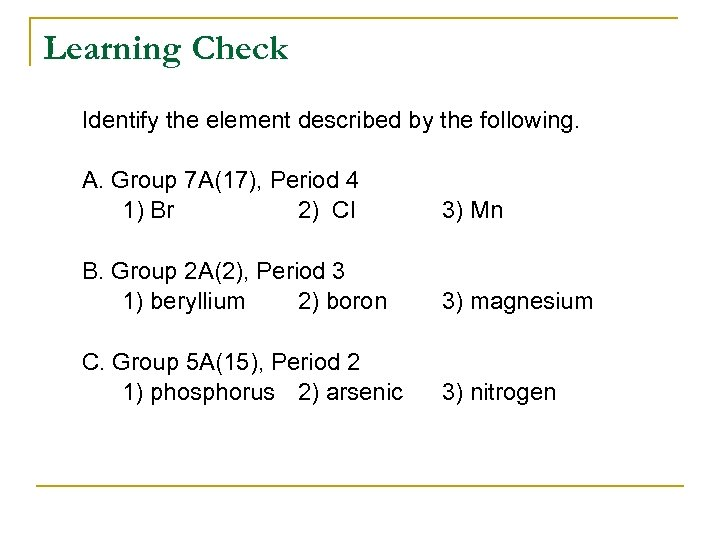Learning Check Identify the element described by the following. A. Group 7 A(17), Period 4 1) Br 2) Cl 3) Mn B. Group 2 A(2), Period 3 1) beryllium 2) boron 3) magnesium C. Group 5 A(15), Period 2 1) phosphorus 2) arsenic 3) nitrogen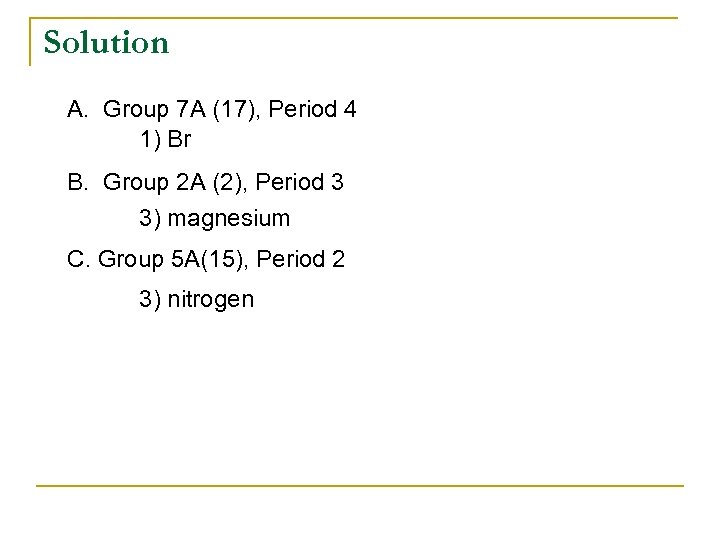Solution A. Group 7 A (17), Period 4 1) Br B. Group 2 A (2), Period 3 3) magnesium C. Group 5 A(15), Period 2 3) nitrogen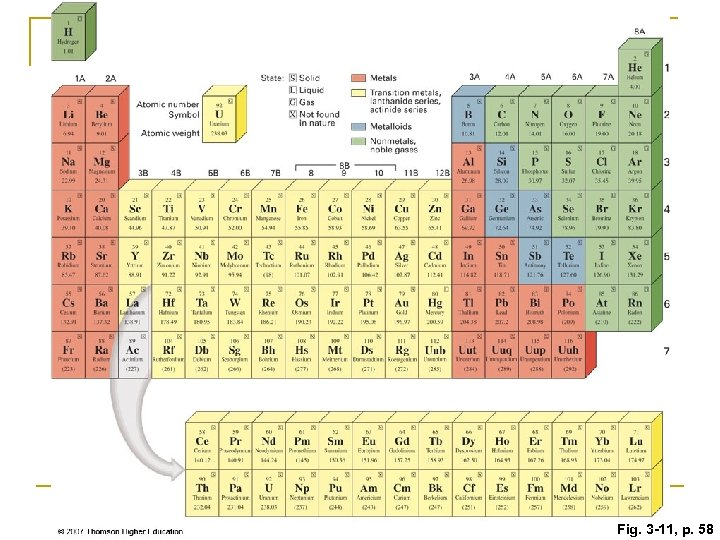Fig. 3 -11, p. 58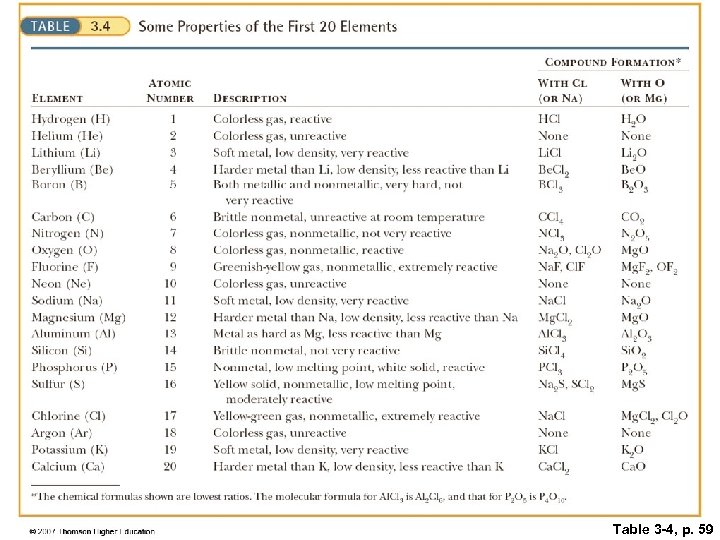Table 3 -4, p. 59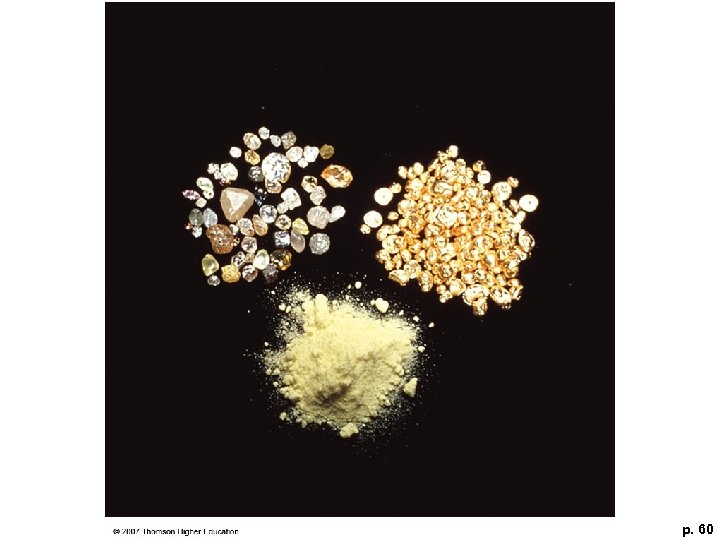p. 60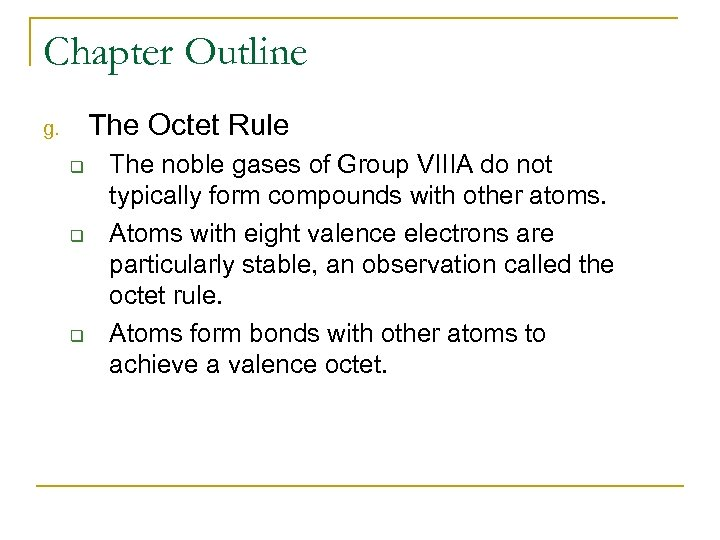Chapter Outline The Octet Rule g. q q q The noble gases of Group VIIIA do not typically form compounds with other atoms. Atoms with eight valence electrons are particularly stable, an observation called the octet rule. Atoms form bonds with other atoms to achieve a valence octet.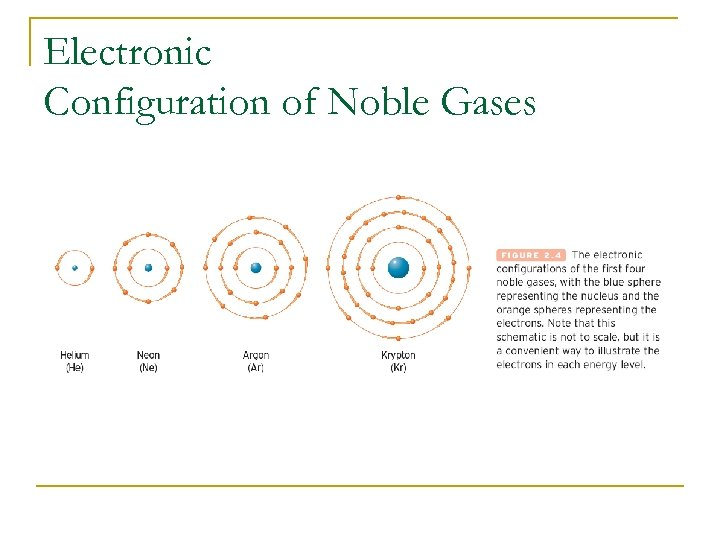Electronic Configuration of Noble Gases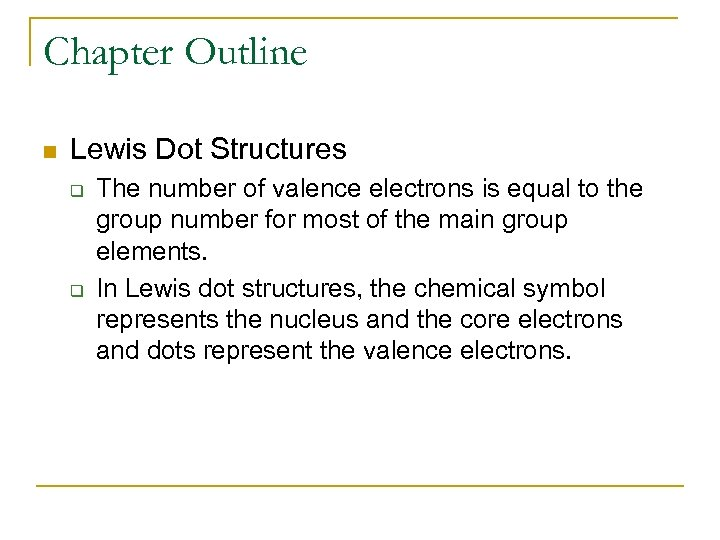Chapter Outline n Lewis Dot Structures q q The number of valence electrons is equal to the group number for most of the main group elements. In Lewis dot structures, the chemical symbol represents the nucleus and the core electrons and dots represent the valence electrons.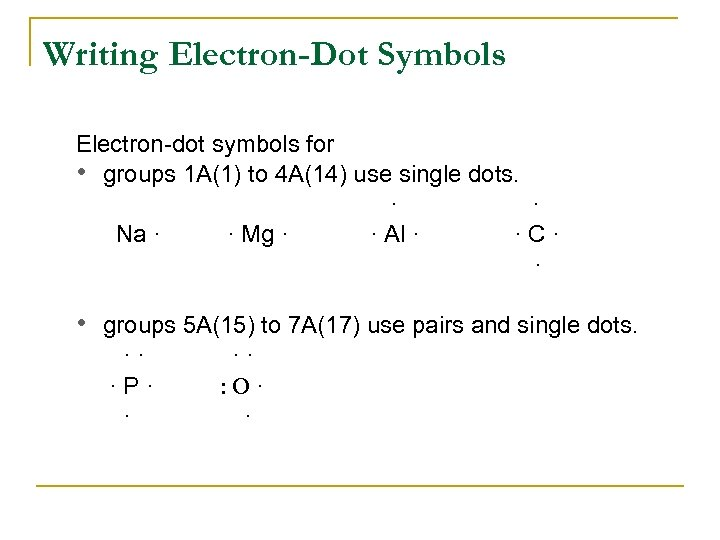Writing Electron-Dot Symbols Electron-dot symbols for • groups 1 A(1) to 4 A(14) use single dots. · · Na · · Mg · · Al · ·C· · • groups 5 A(15) to 7 A(17) use pairs and single dots. ·· ·P· · ·· : O· ·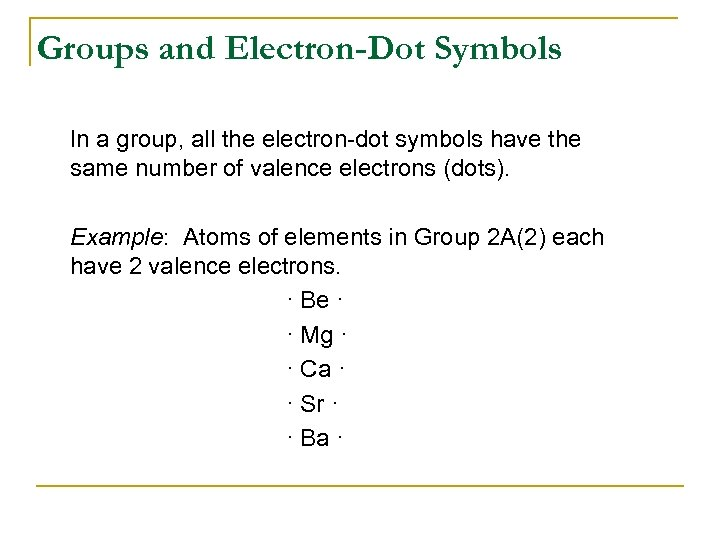Groups and Electron-Dot Symbols In a group, all the electron-dot symbols have the same number of valence electrons (dots). Example: Atoms of elements in Group 2 A(2) each have 2 valence electrons. · Be · · Mg · · Ca · · Sr · · Ba ·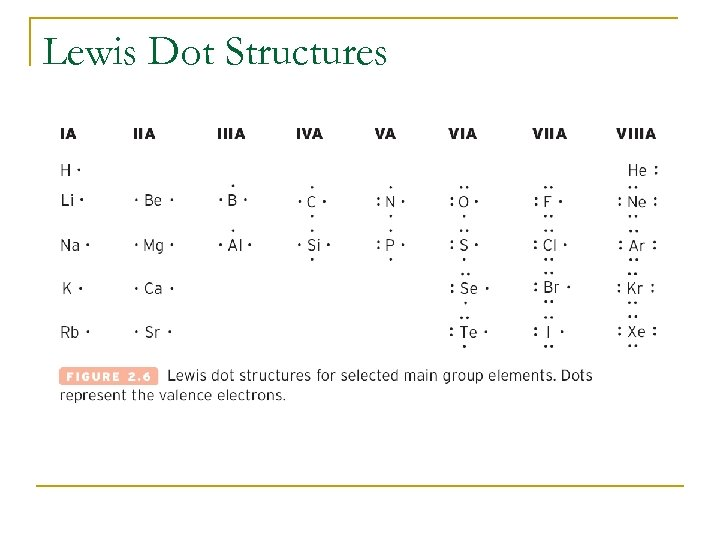Lewis Dot Structures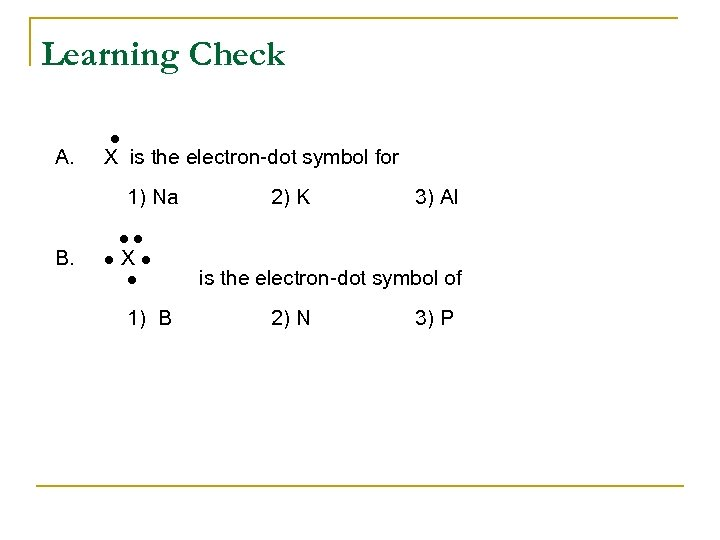Learning Check A. X is the electron-dot symbol for 1) Na B. 2) K 3) Al X 1) B is the electron-dot symbol of 2) N 3) P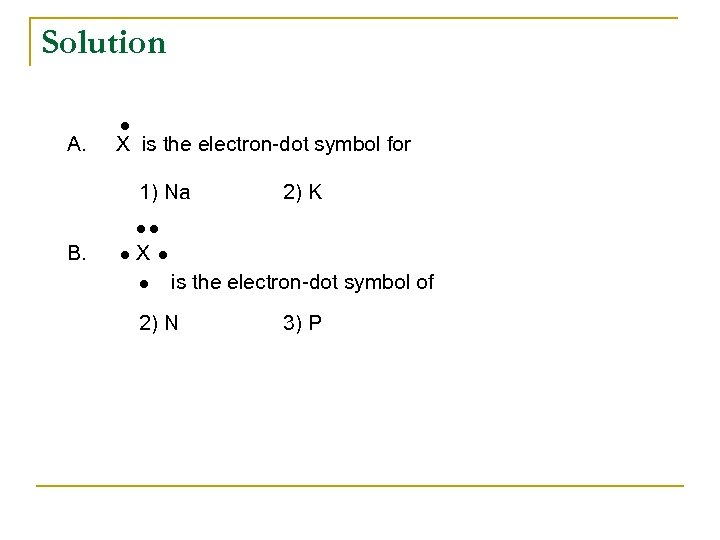Solution A. X is the electron-dot symbol for 1) Na 2) K B. X is the electron-dot symbol of 2) N 3) P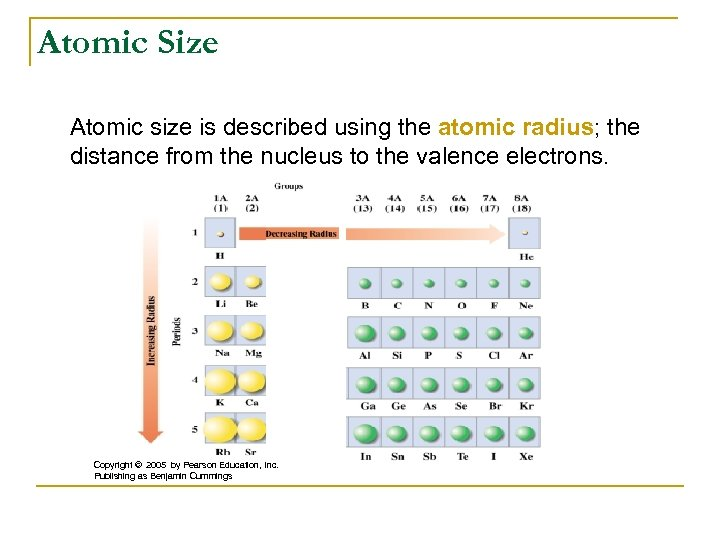Atomic Size Atomic size is described using the atomic radius; the distance from the nucleus to the valence electrons. Copyright © 2005 by Pearson Education, Inc. Publishing as Benjamin Cummings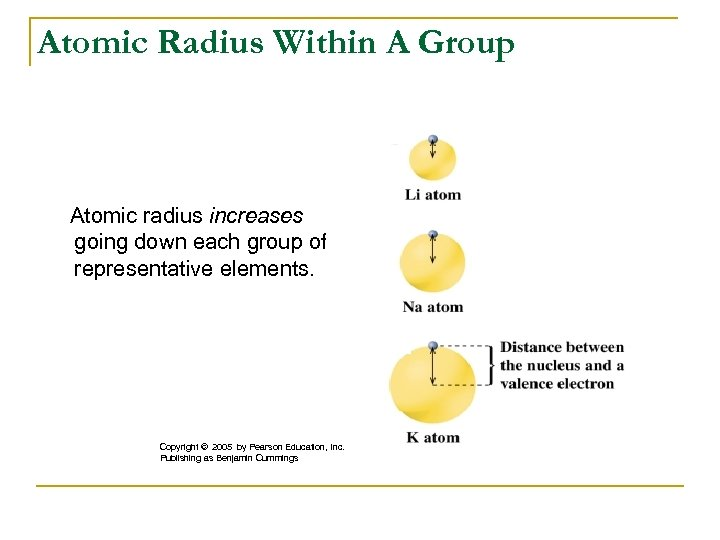Atomic Radius Within A Group Atomic radius increases going down each group of representative elements. Copyright © 2005 by Pearson Education, Inc. Publishing as Benjamin Cummings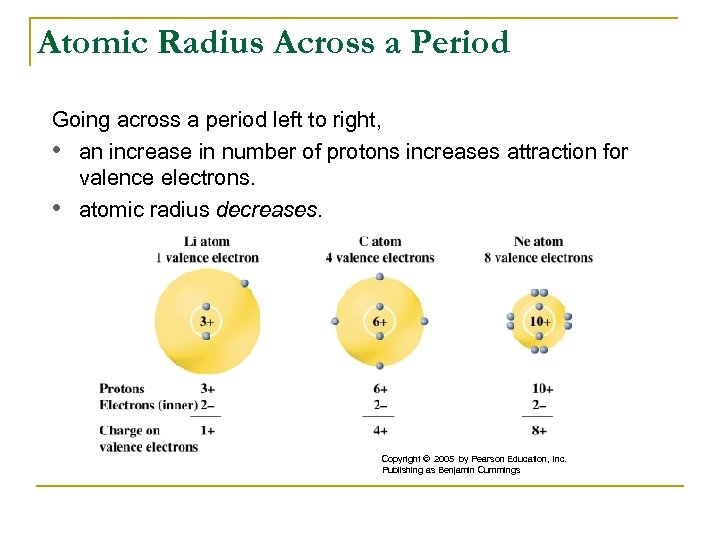Atomic Radius Across a Period Going across a period left to right, • an increase in number of protons increases attraction for valence electrons. • atomic radius decreases. Copyright © 2005 by Pearson Education, Inc. Publishing as Benjamin Cummings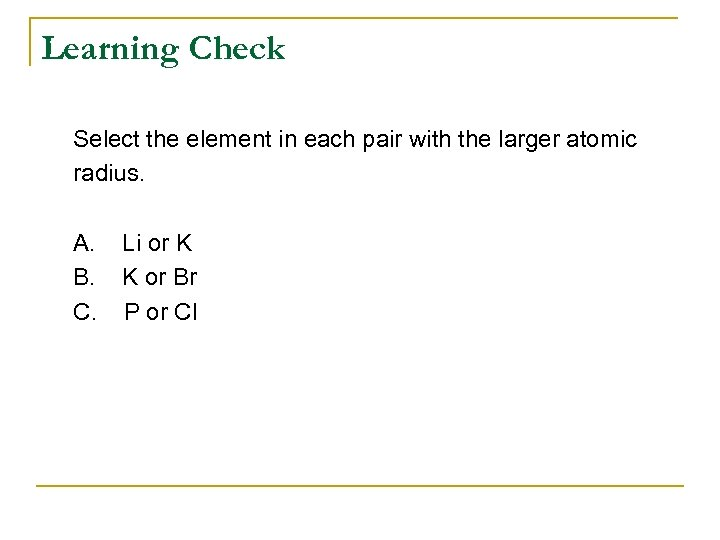Learning Check Select the element in each pair with the larger atomic radius. A. B. C. Li or K K or Br P or Cl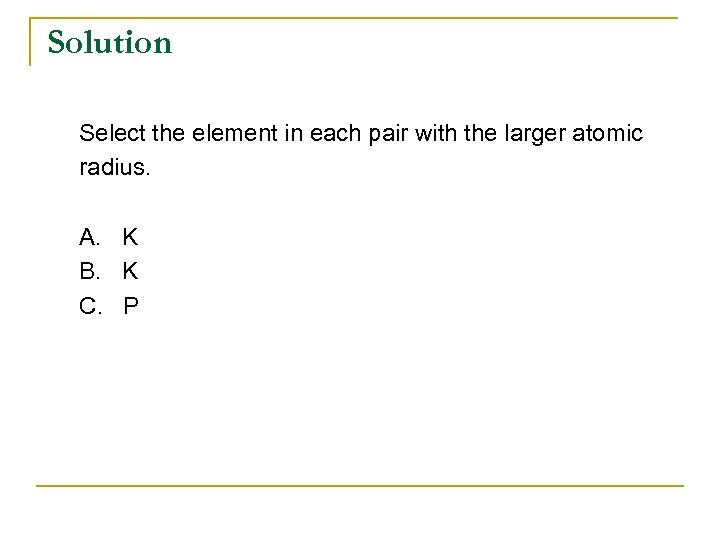Solution Select the element in each pair with the larger atomic radius. A. K B. K C. P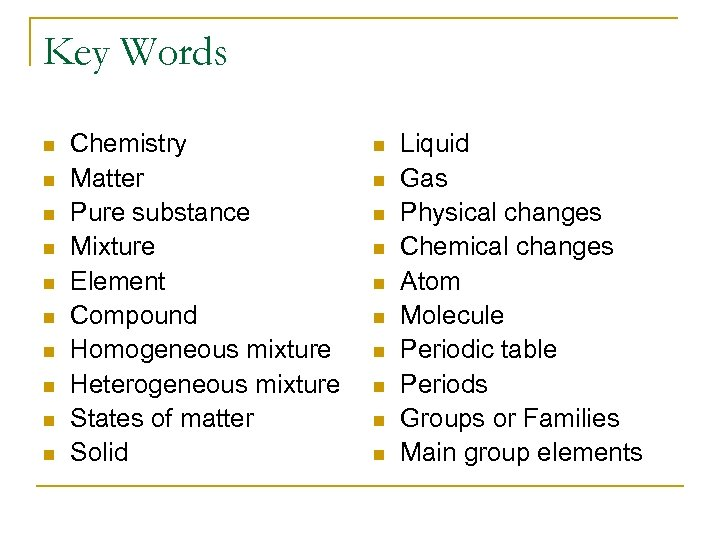Key Words n n n n n Chemistry Matter Pure substance Mixture Element Compound Homogeneous mixture Heterogeneous mixture States of matter Solid n n n n n Liquid Gas Physical changes Chemical changes Atom Molecule Periodic table Periods Groups or Families Main group elements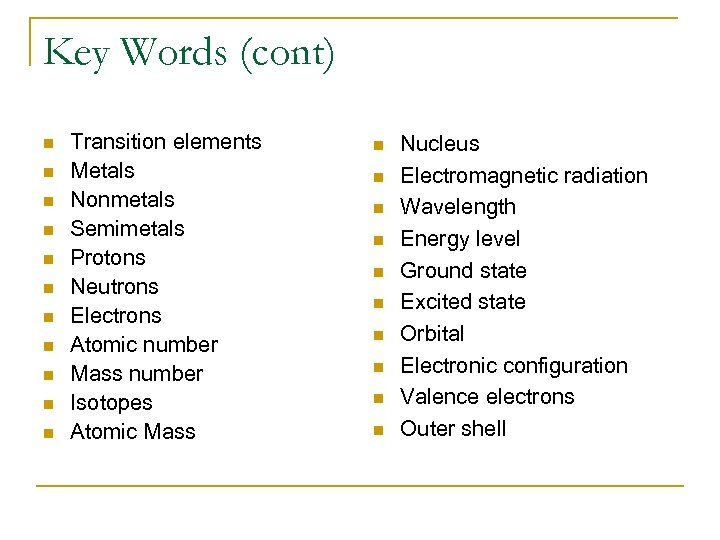Key Words (cont) n n n Transition elements Metals Nonmetals Semimetals Protons Neutrons Electrons Atomic number Mass number Isotopes Atomic Mass n n n n n Nucleus Electromagnetic radiation Wavelength Energy level Ground state Excited state Orbital Electronic configuration Valence electrons Outer shell